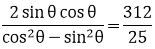# RD Sharma Solutions for Class 10 Maths Chapter 5 Trigonometric Ratios Exercise 5.1

The science of measuring triangles is trigonometry. In other words, it deals with the measurement of sides and angles of triangles. This exercise contains problems dealing with finding all the trigonometric ratios when one of it is given. Further, the value of an expression is also asked to be evaluated. All the solutions to this and other chapters are easily available on the RD Sharma Solutions Class 10. For specific doubts regarding the solution and concept, students can download RD Sharma Solutions for Class 10 Maths Chapter 5 Trigonometric Ratios Exercise 5.1 PDF below.

## RD Sharma Solutions for Class 10 Maths Chapter 5 Trigonometric Ratios Exercise 5.1 Download PDF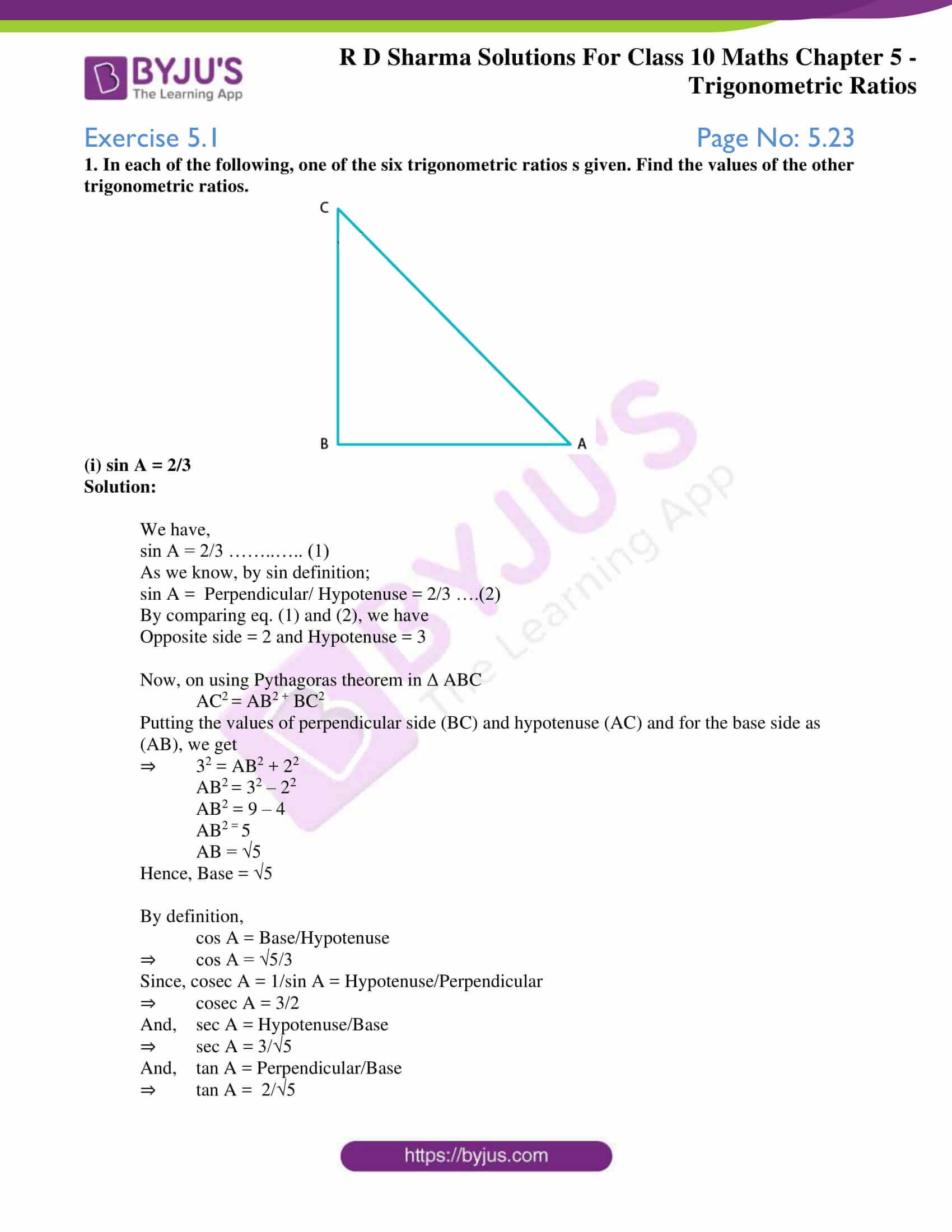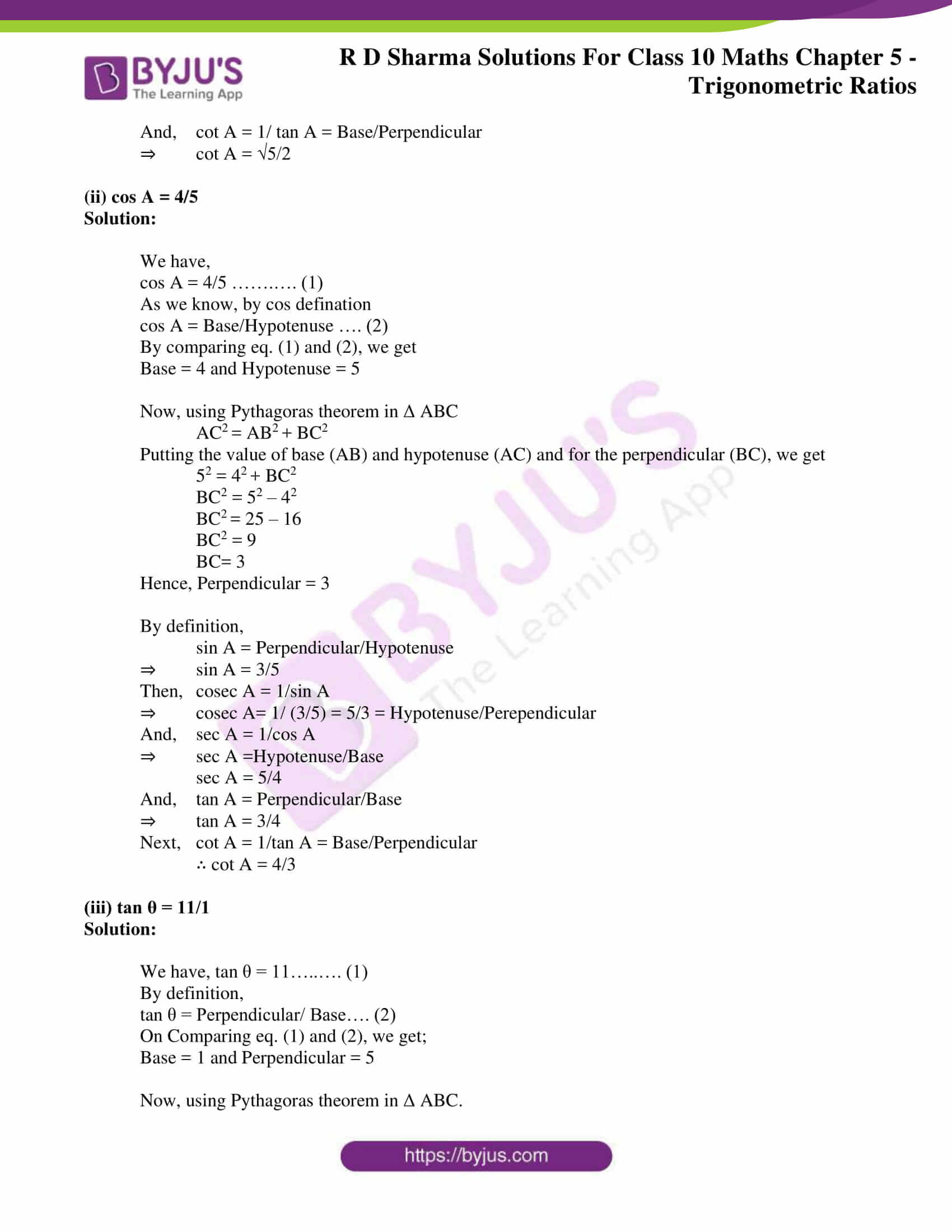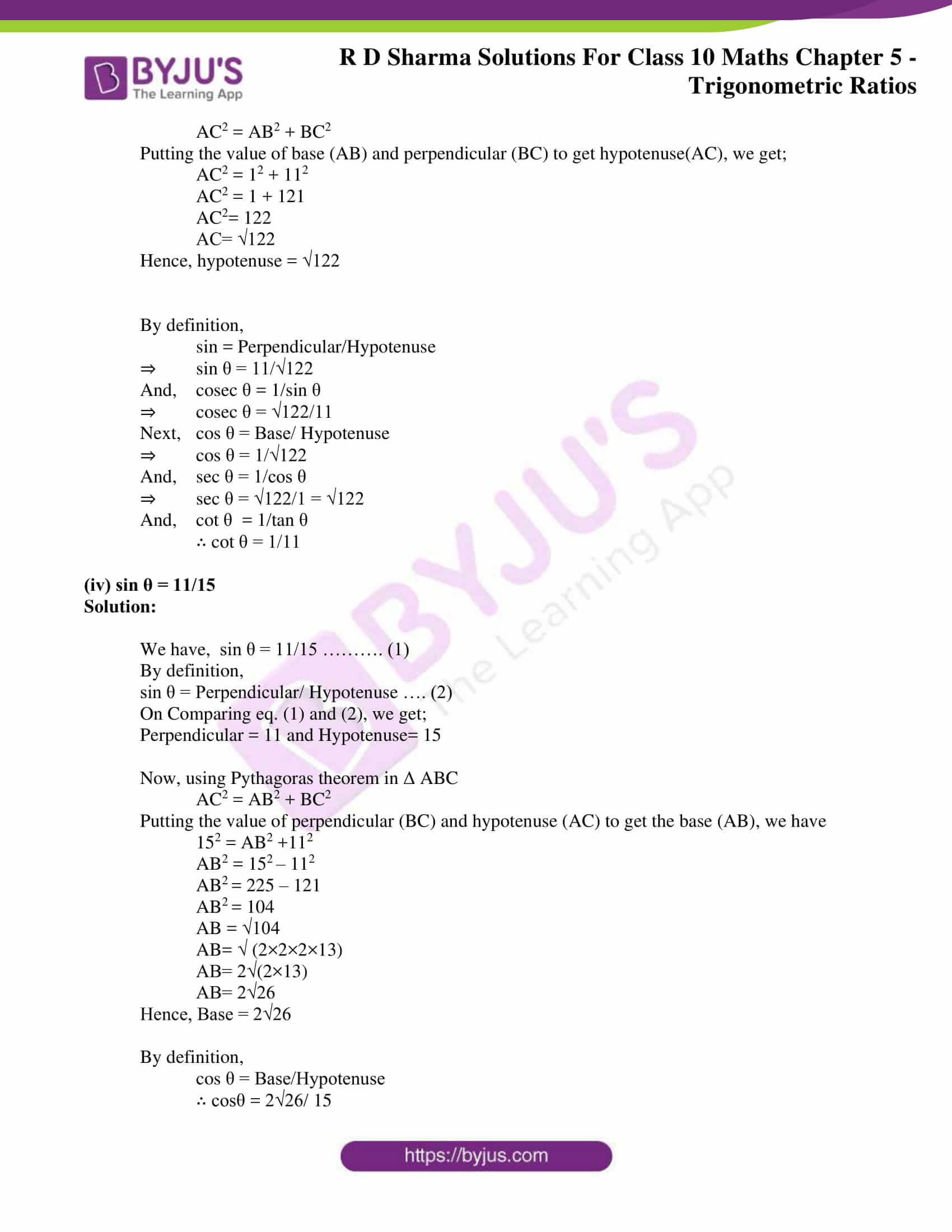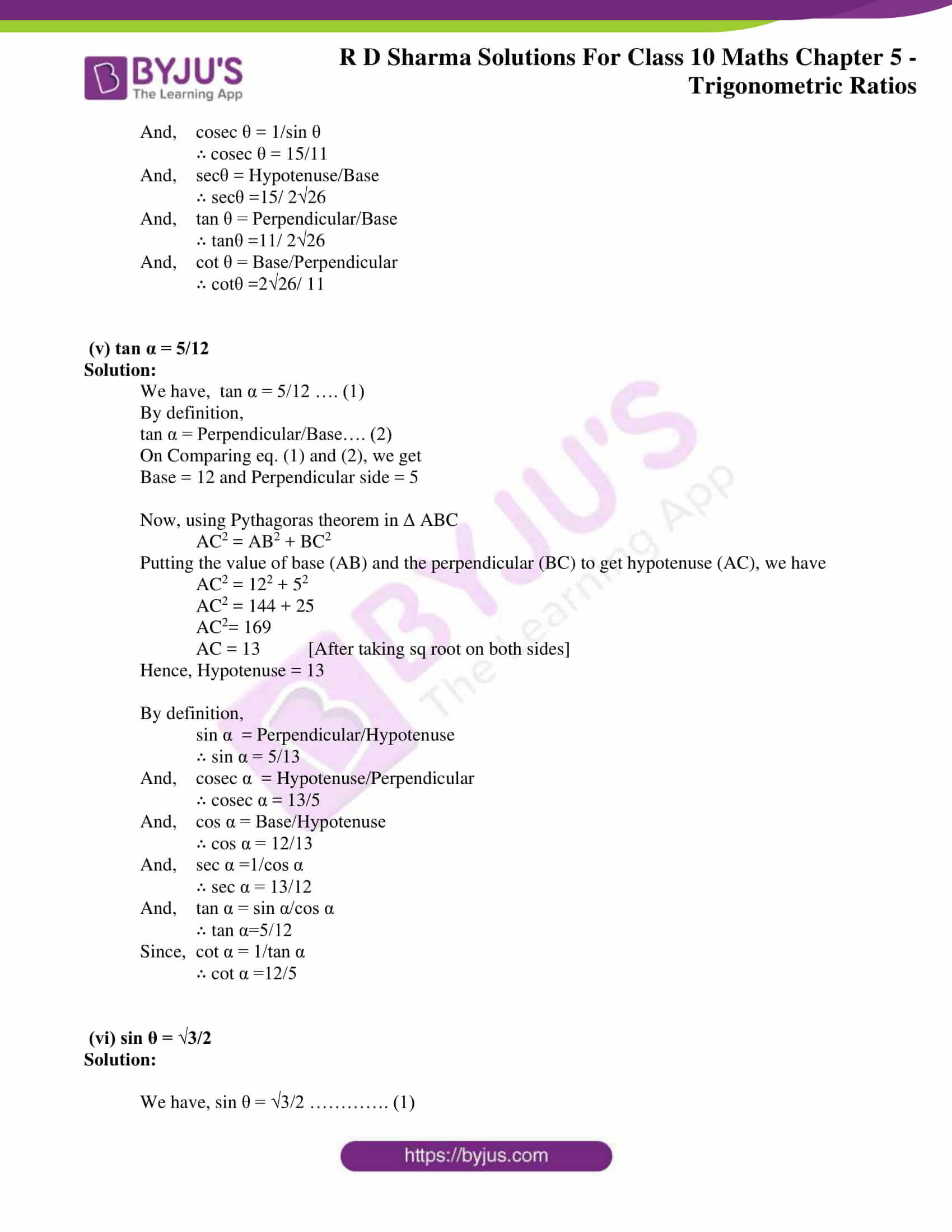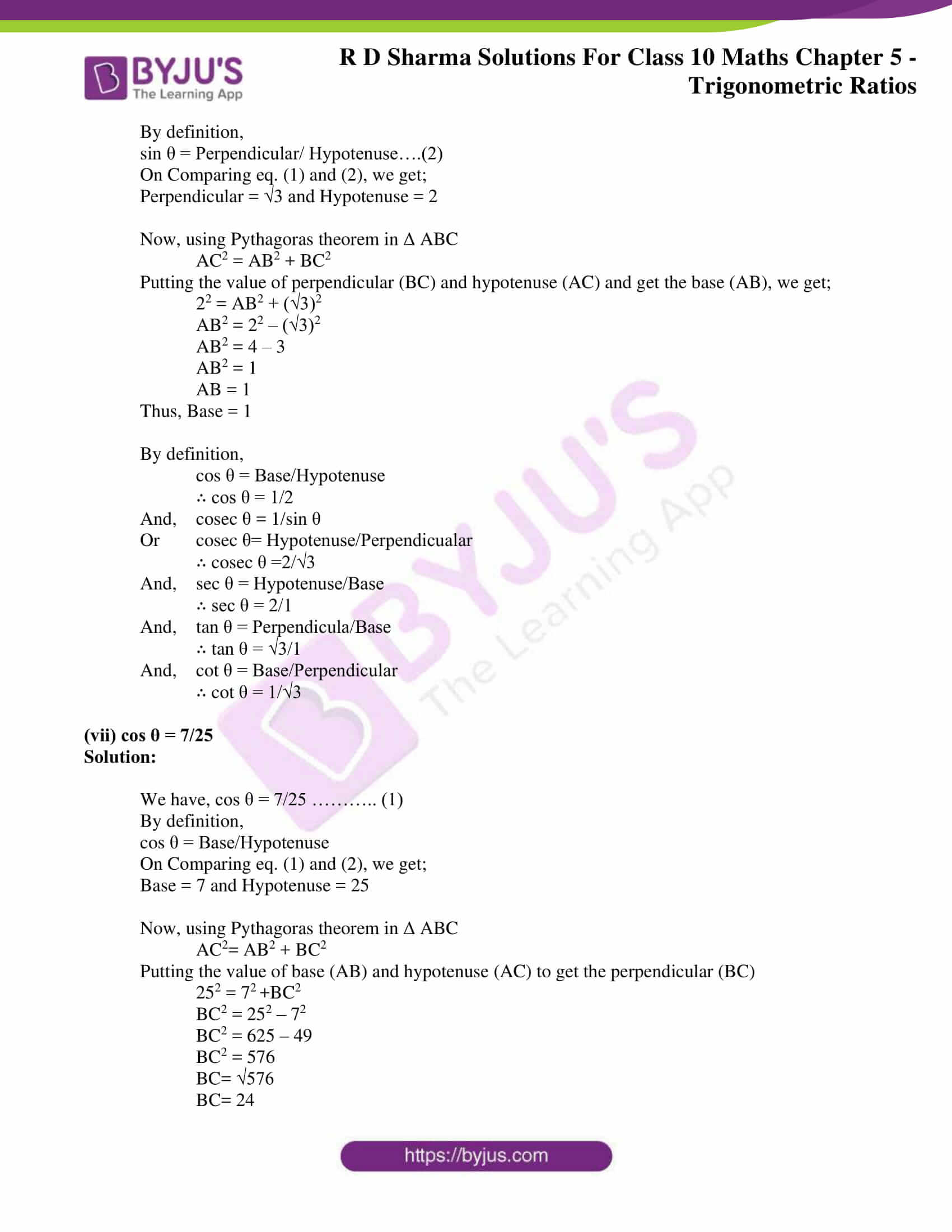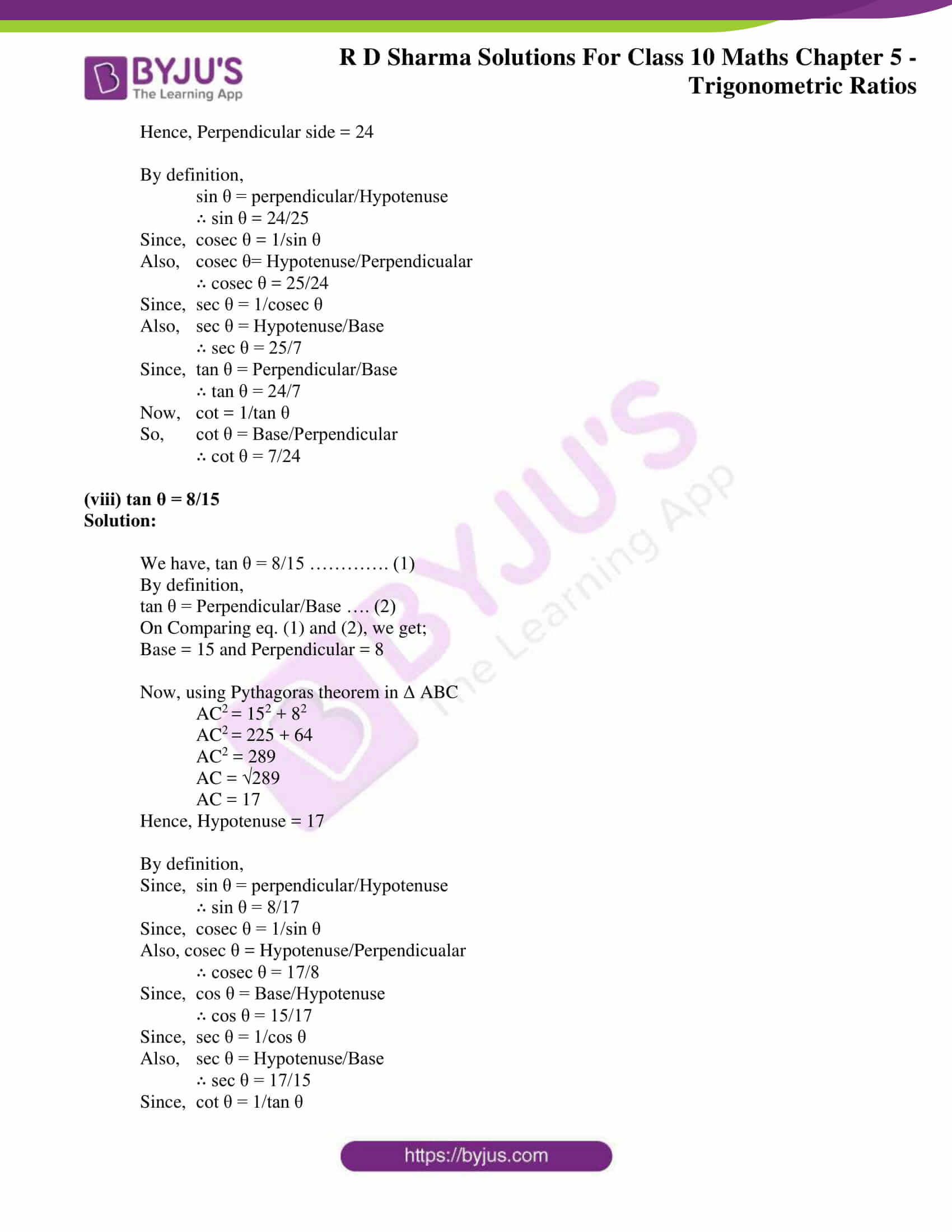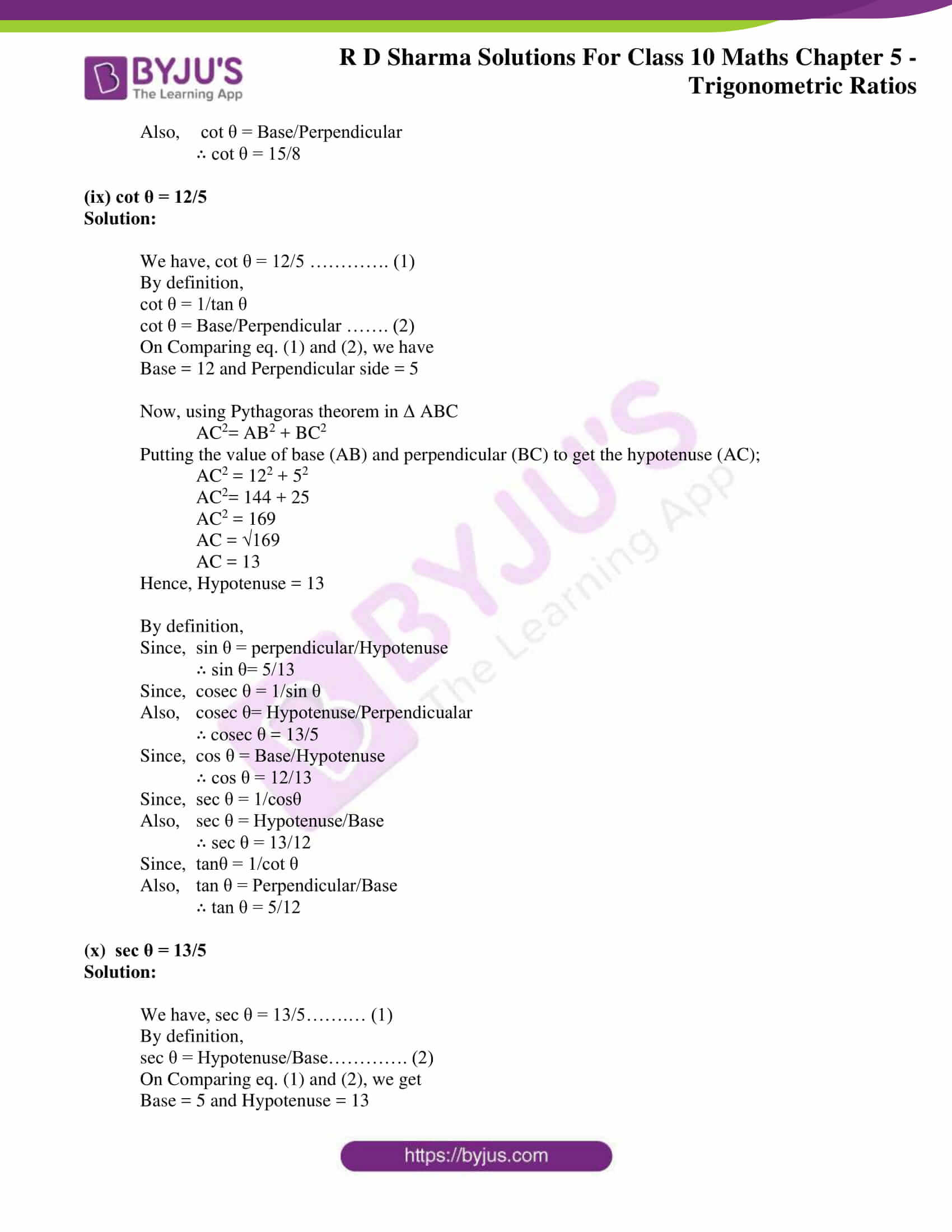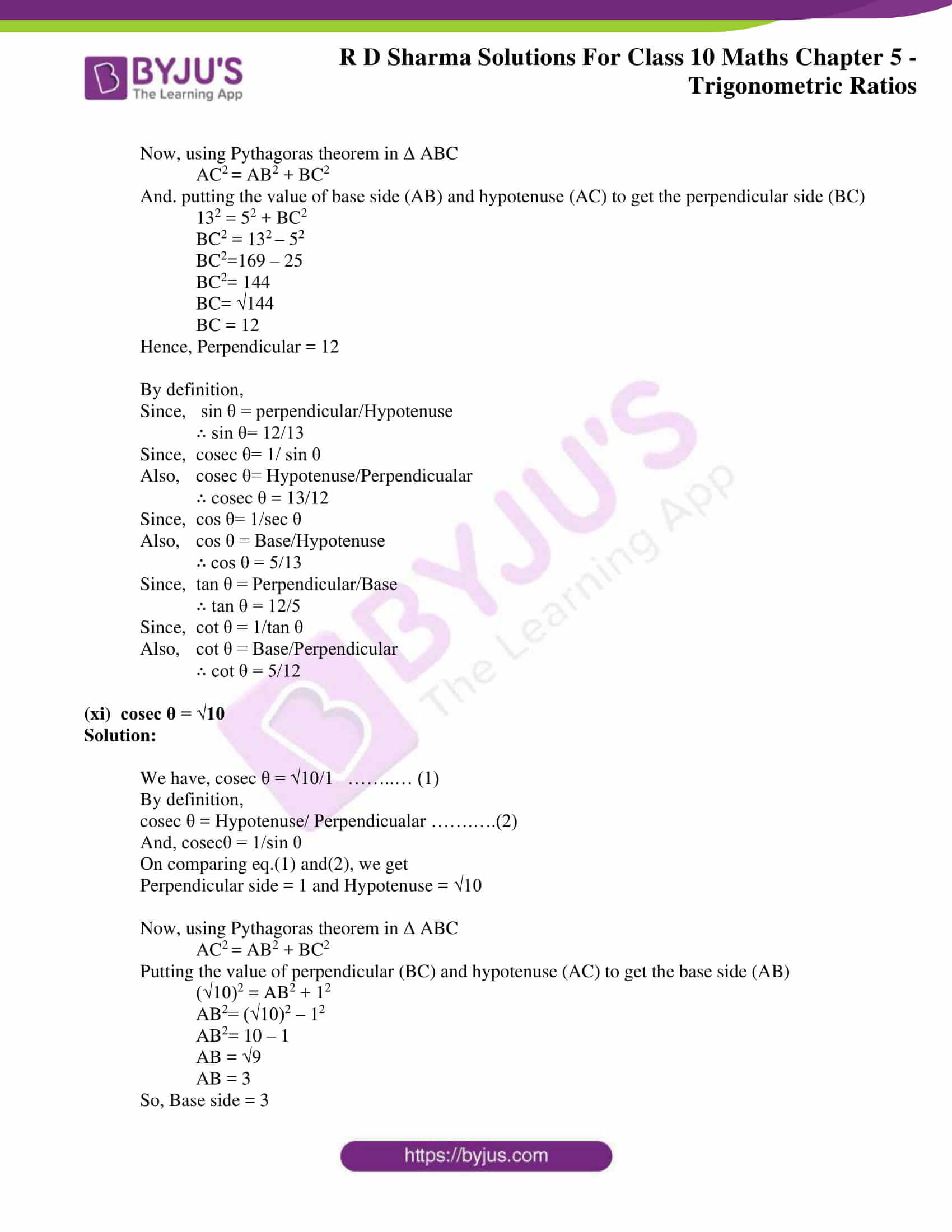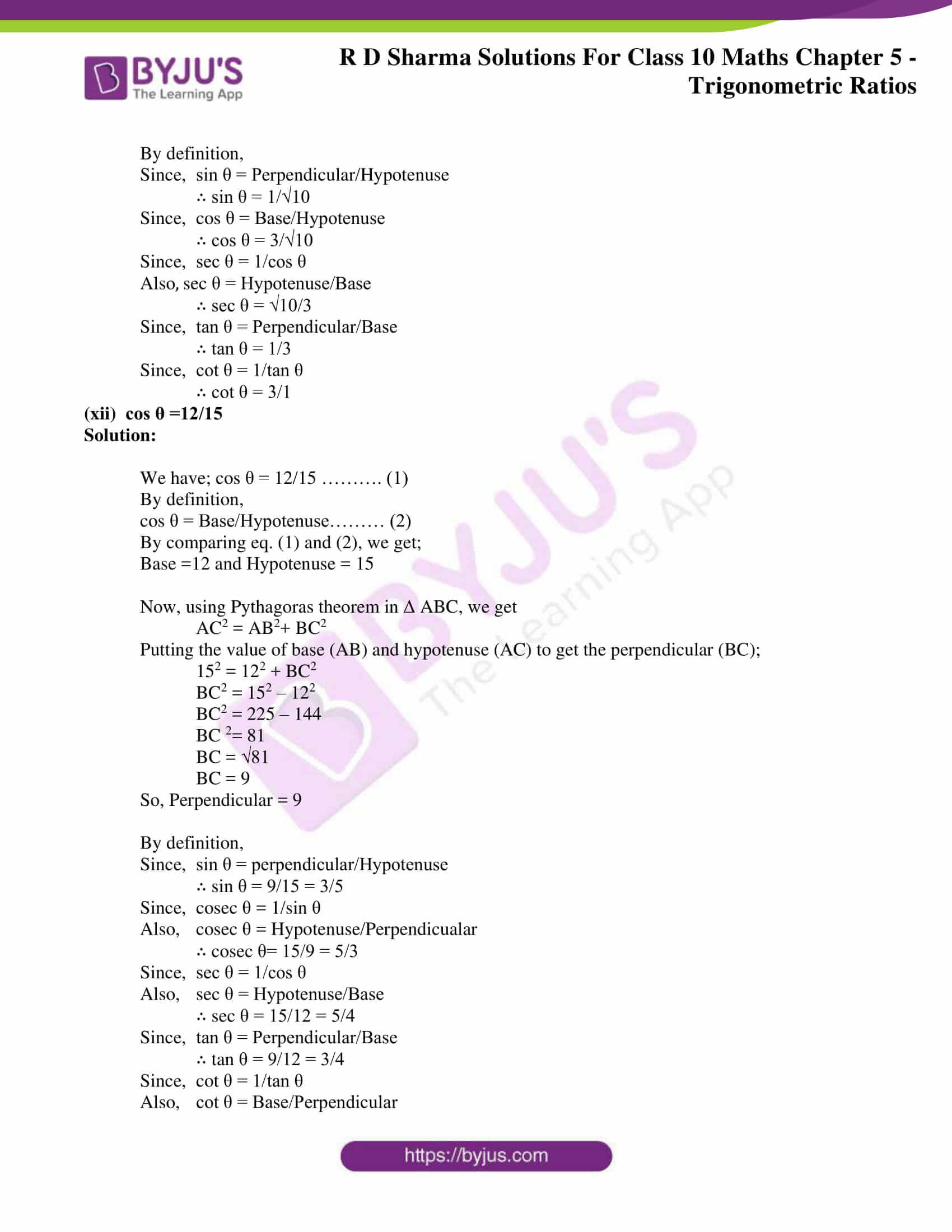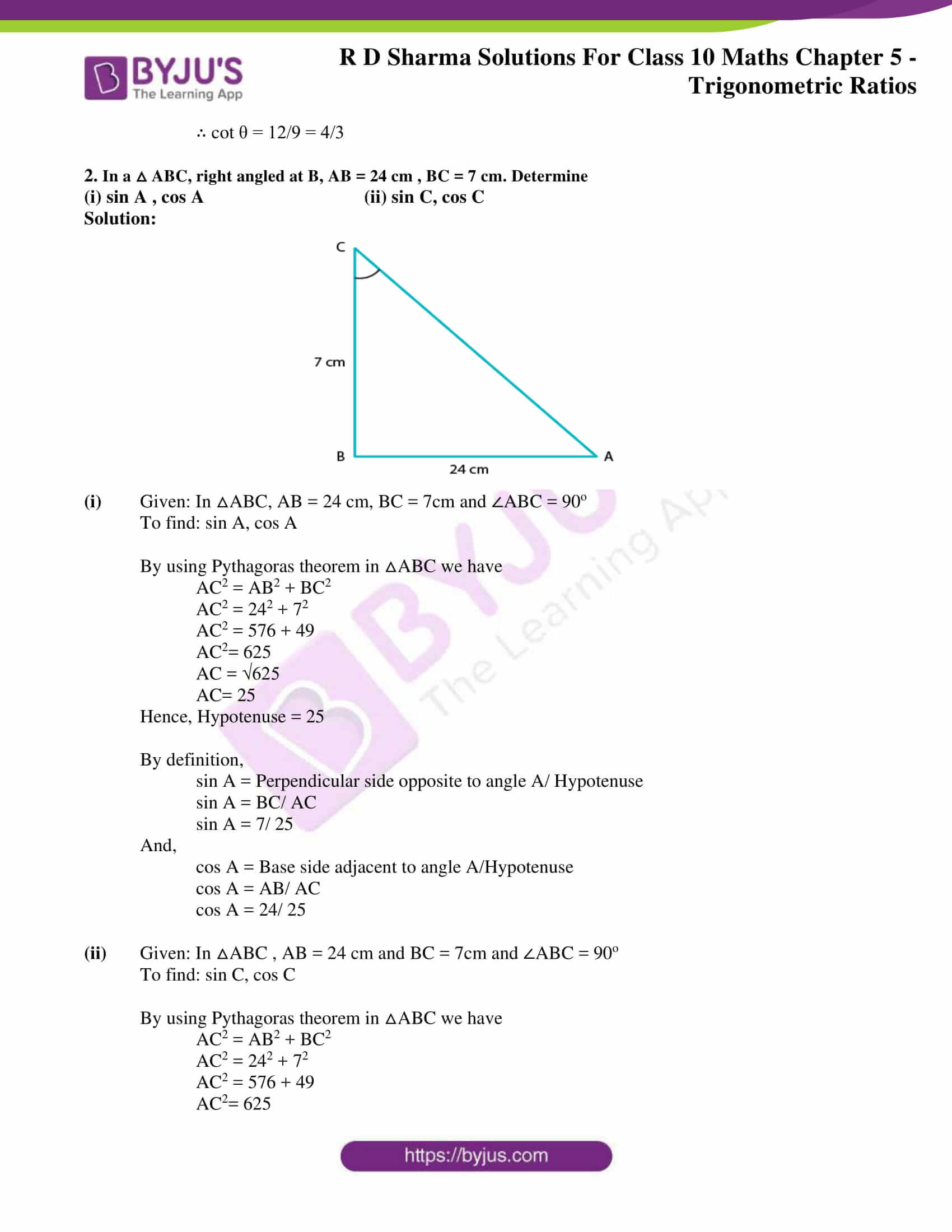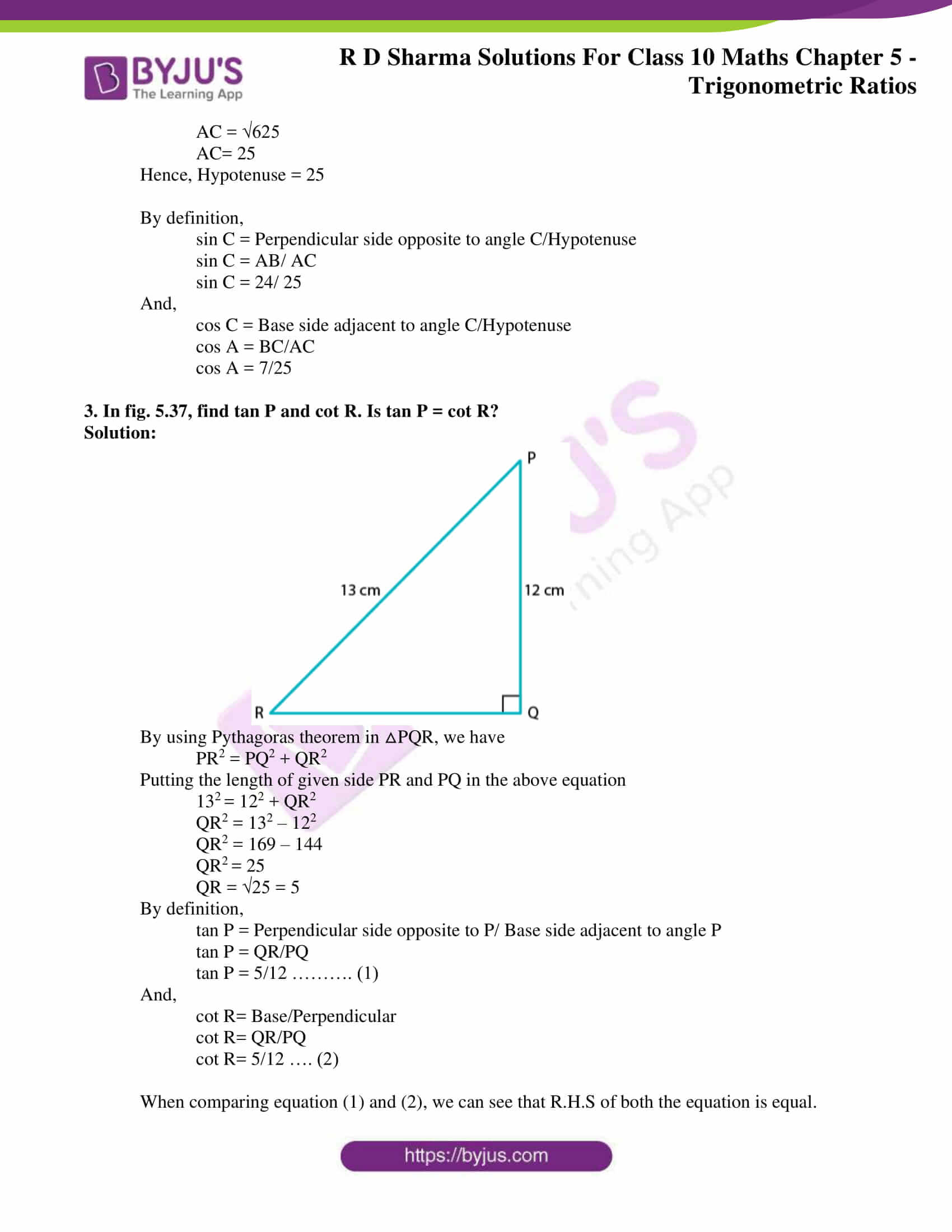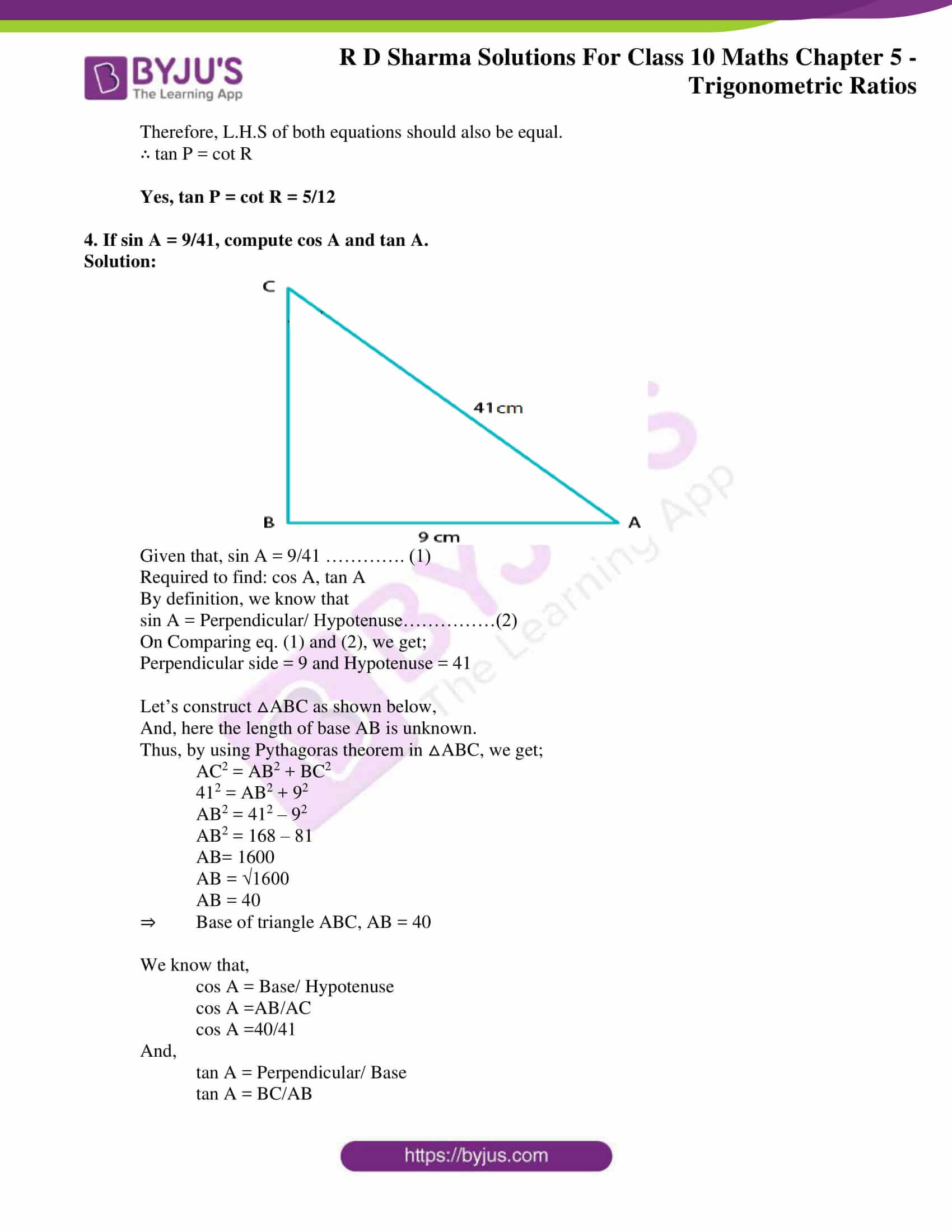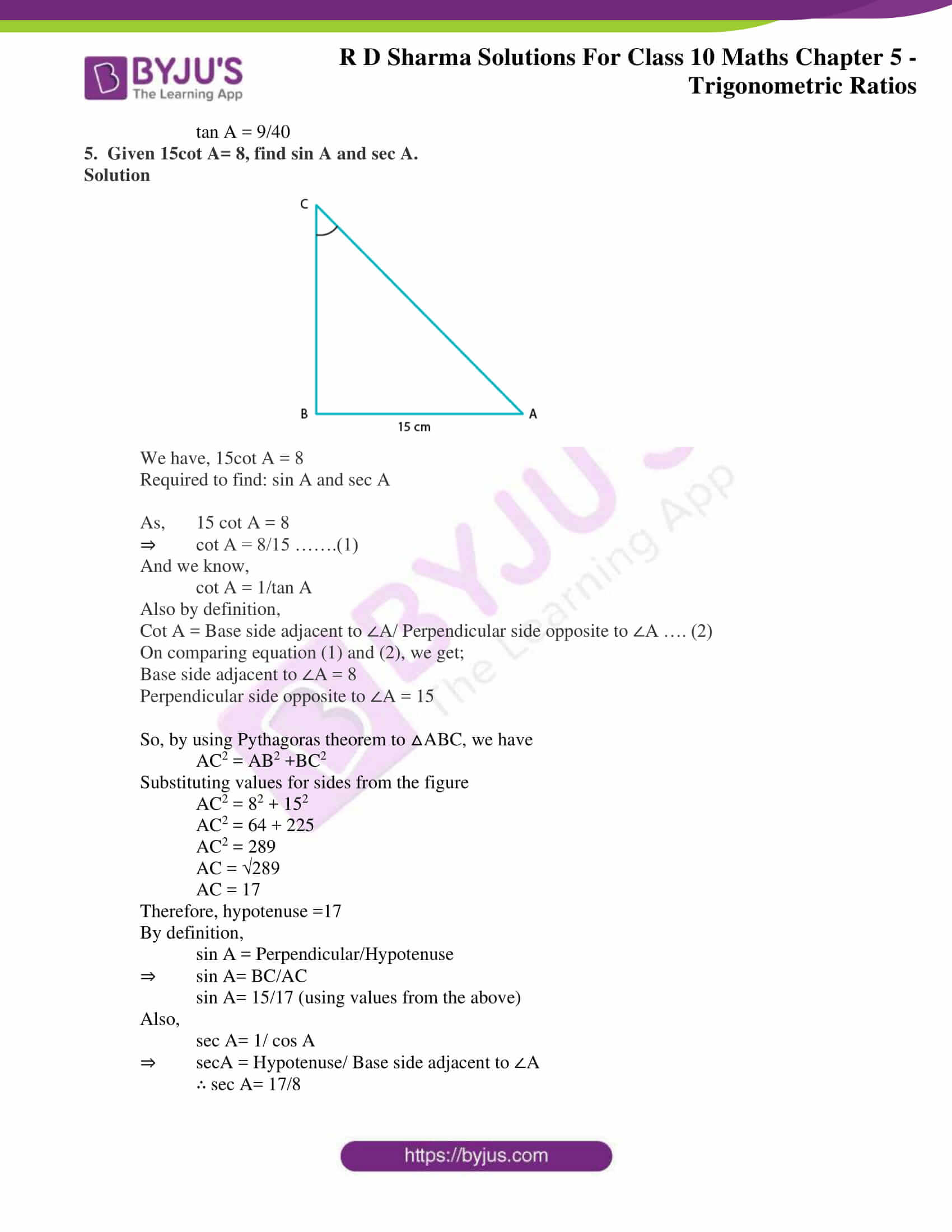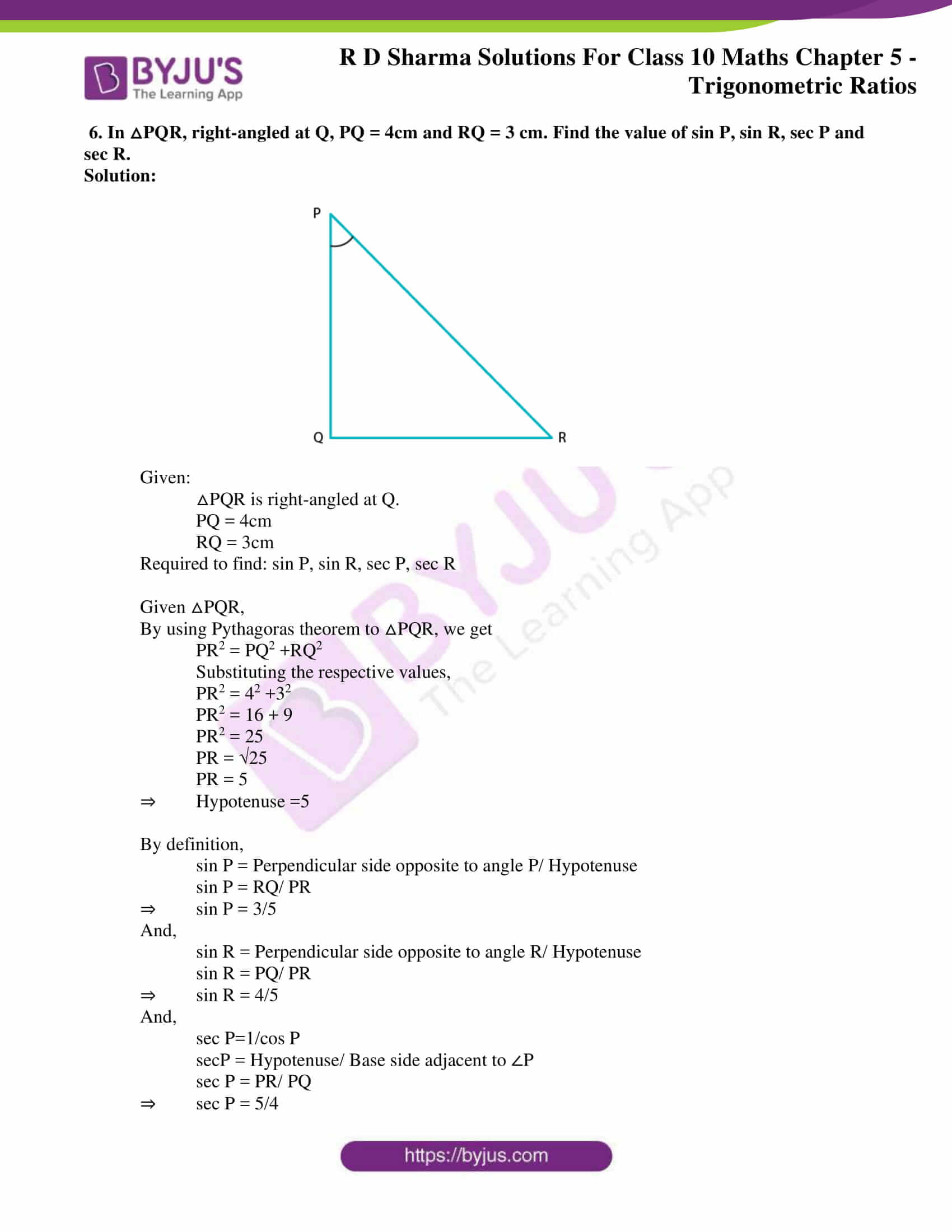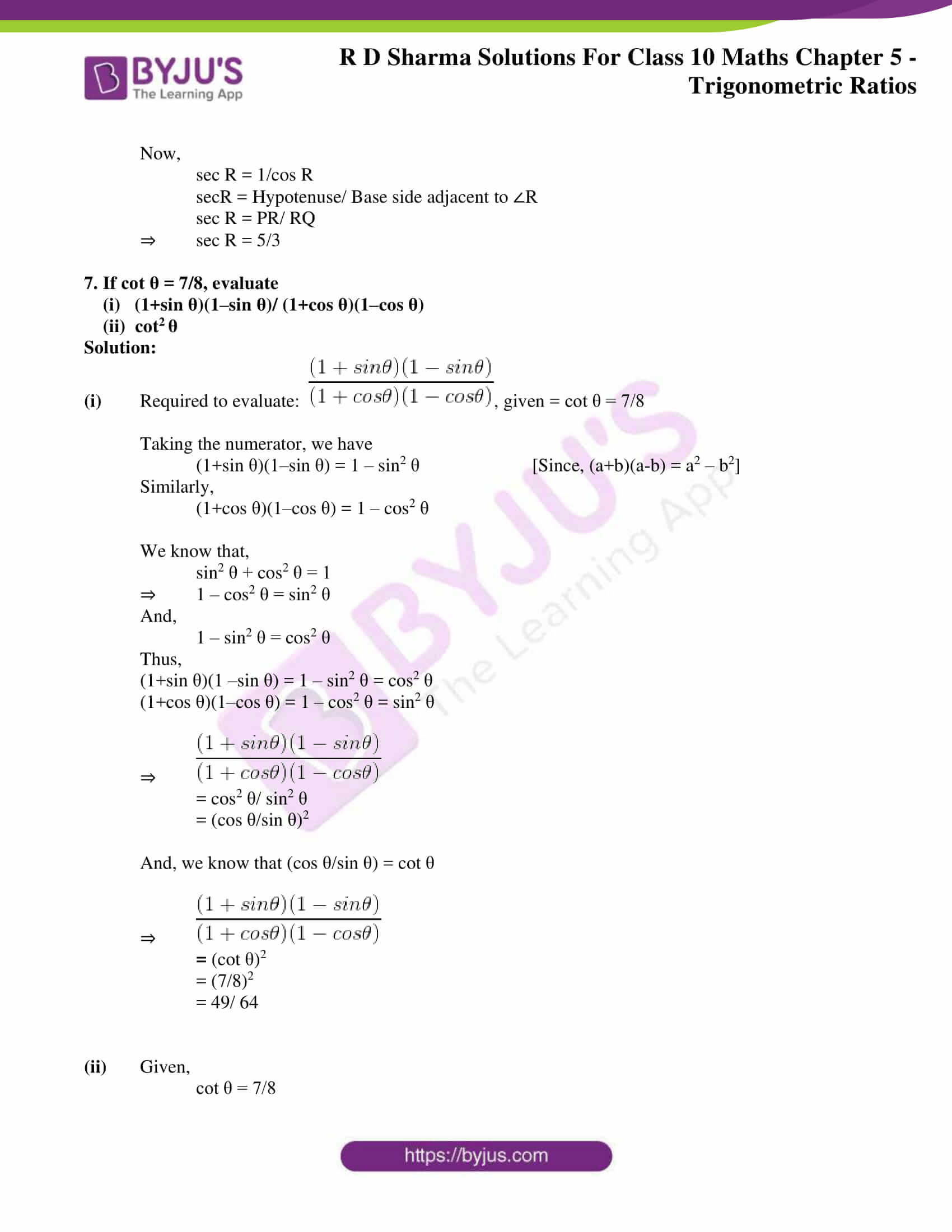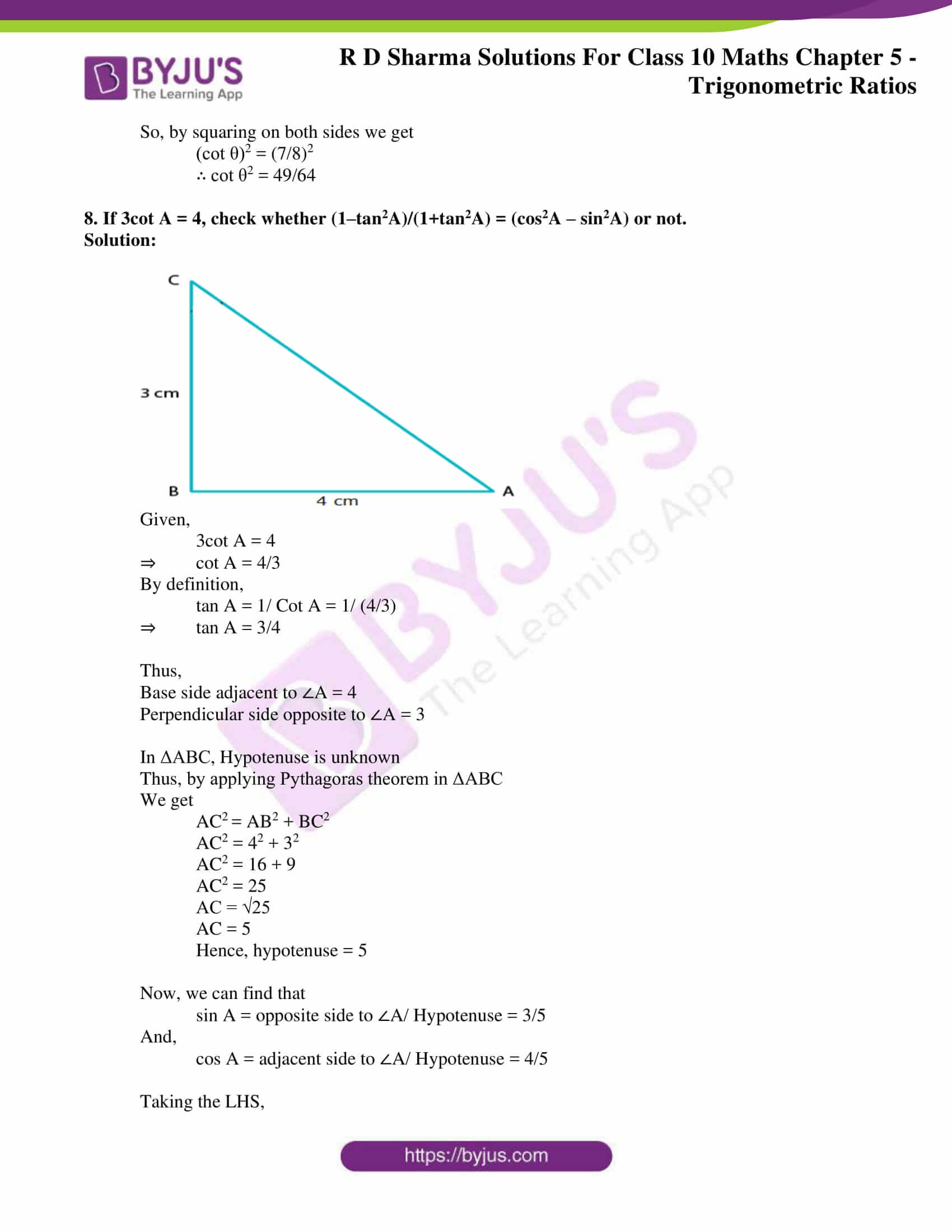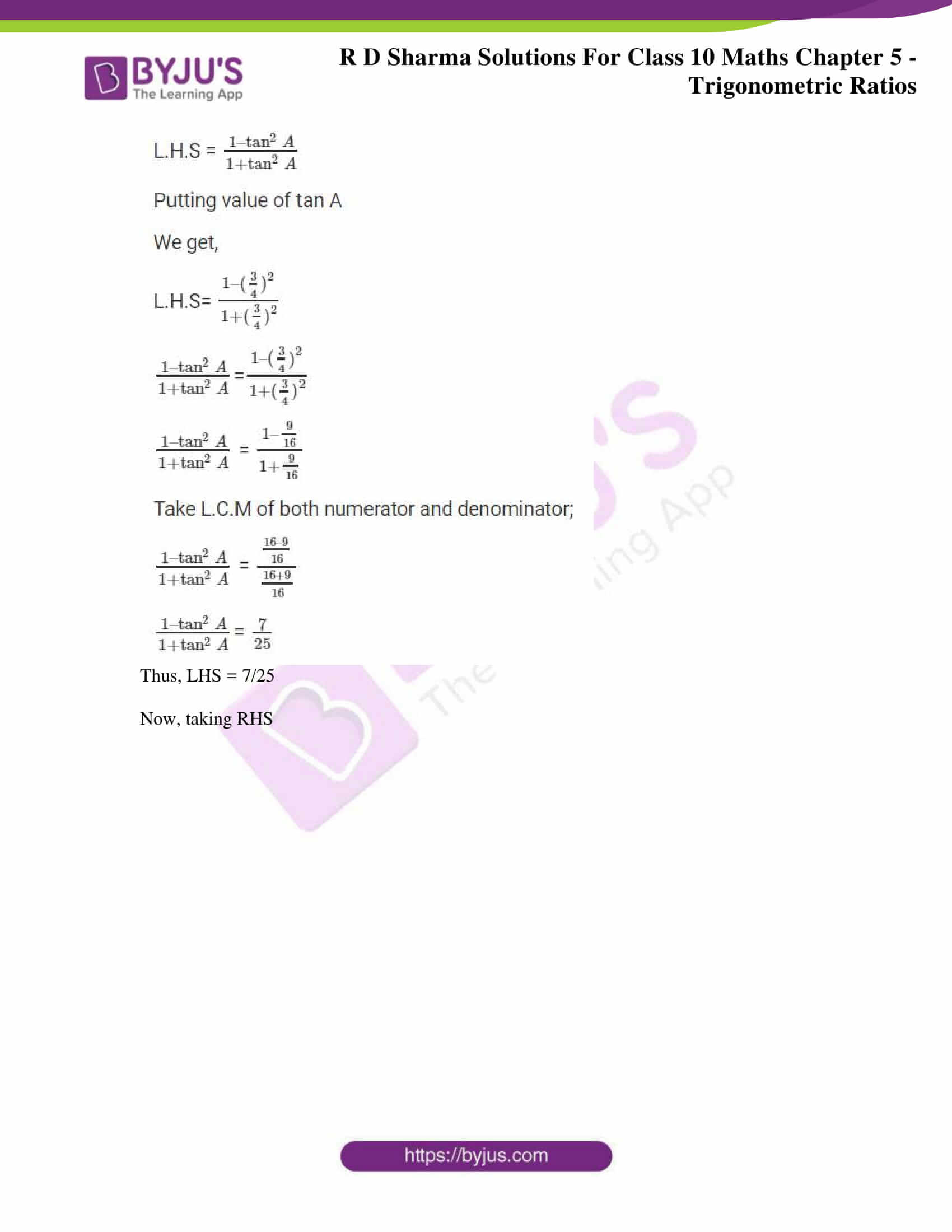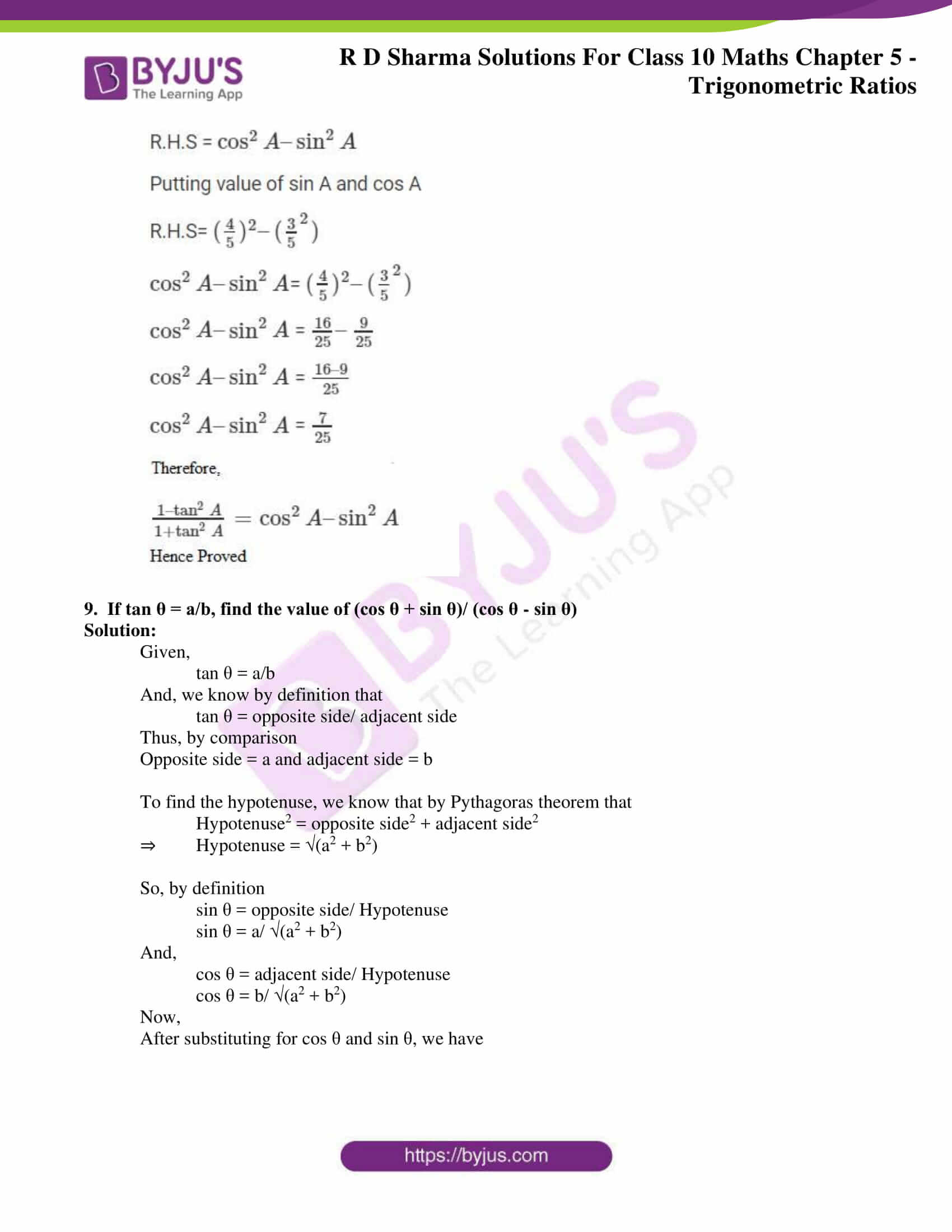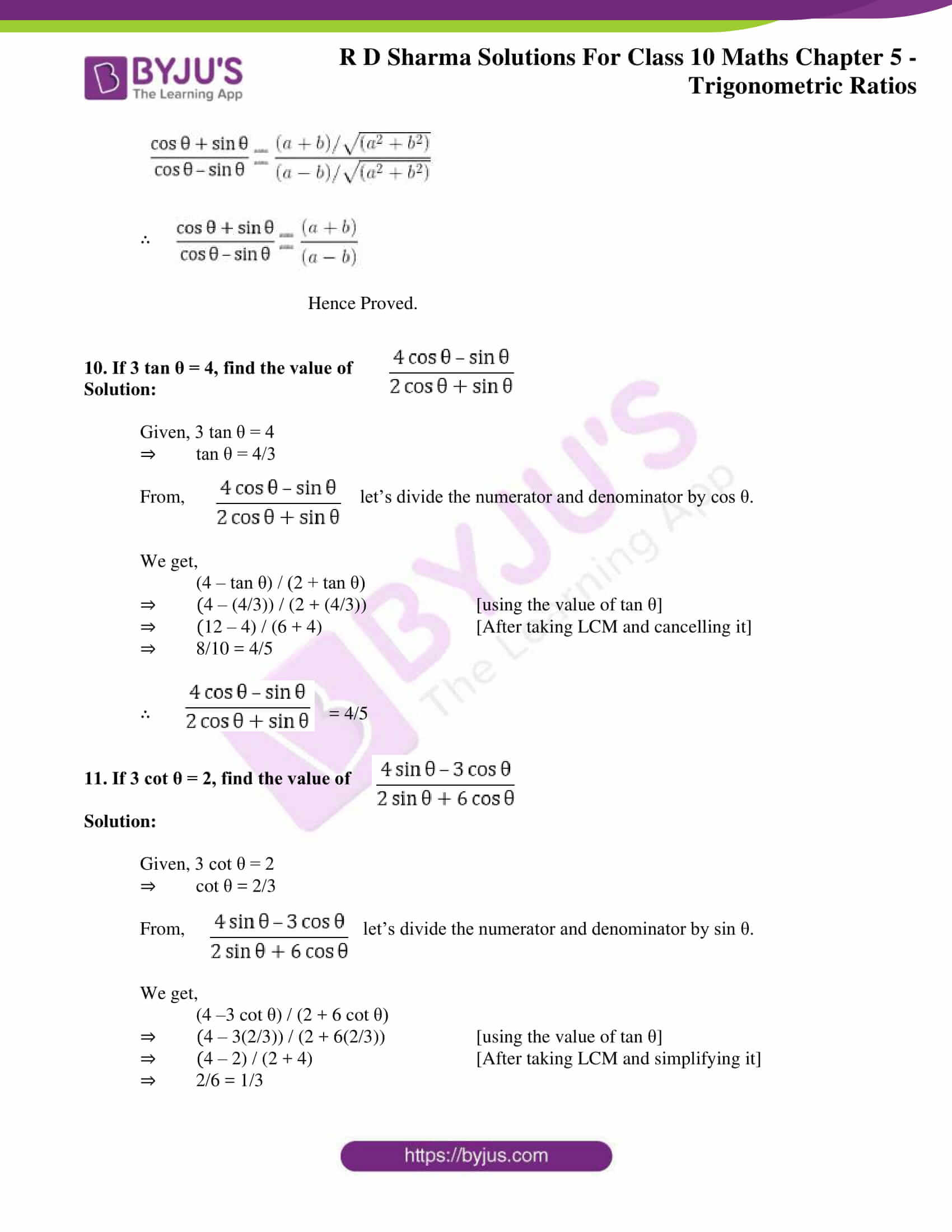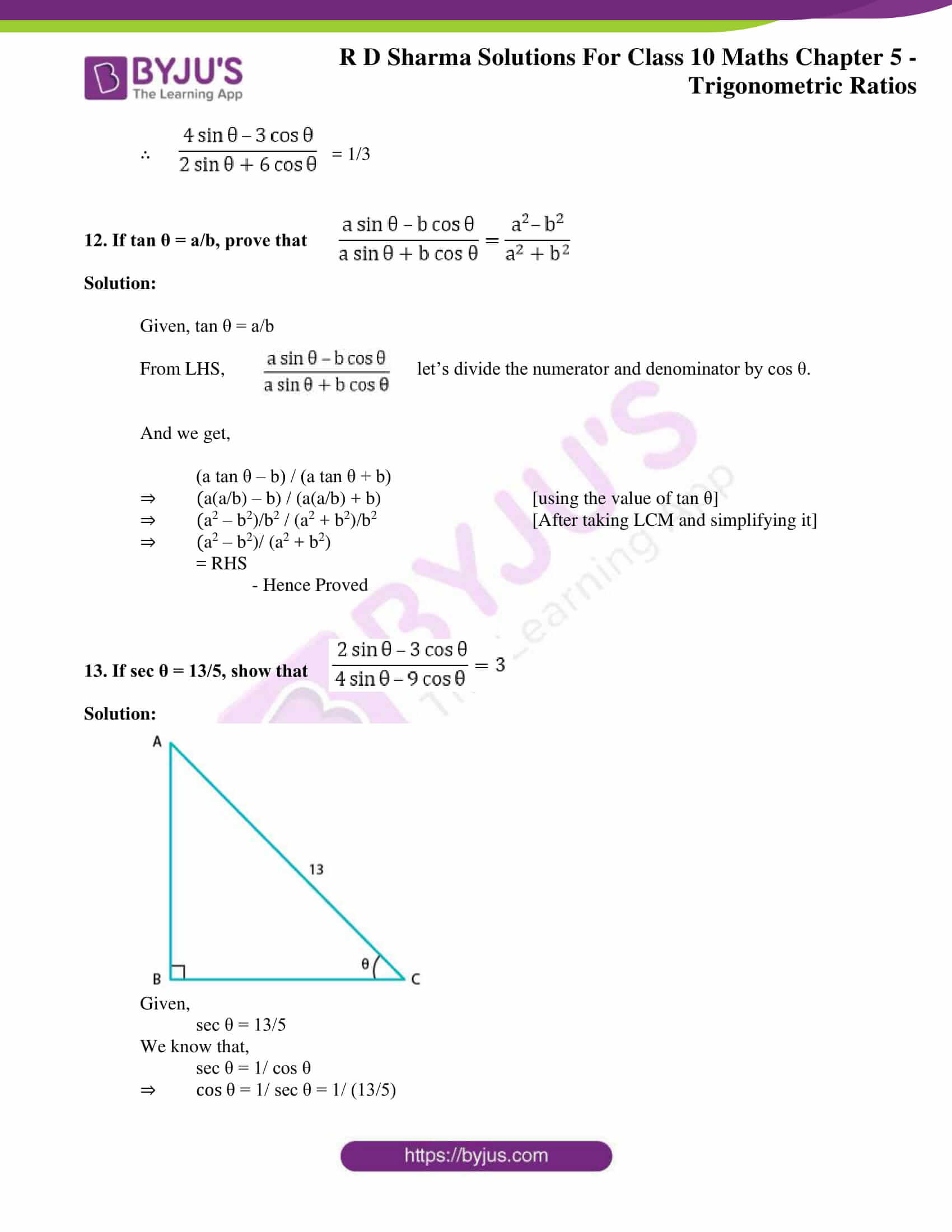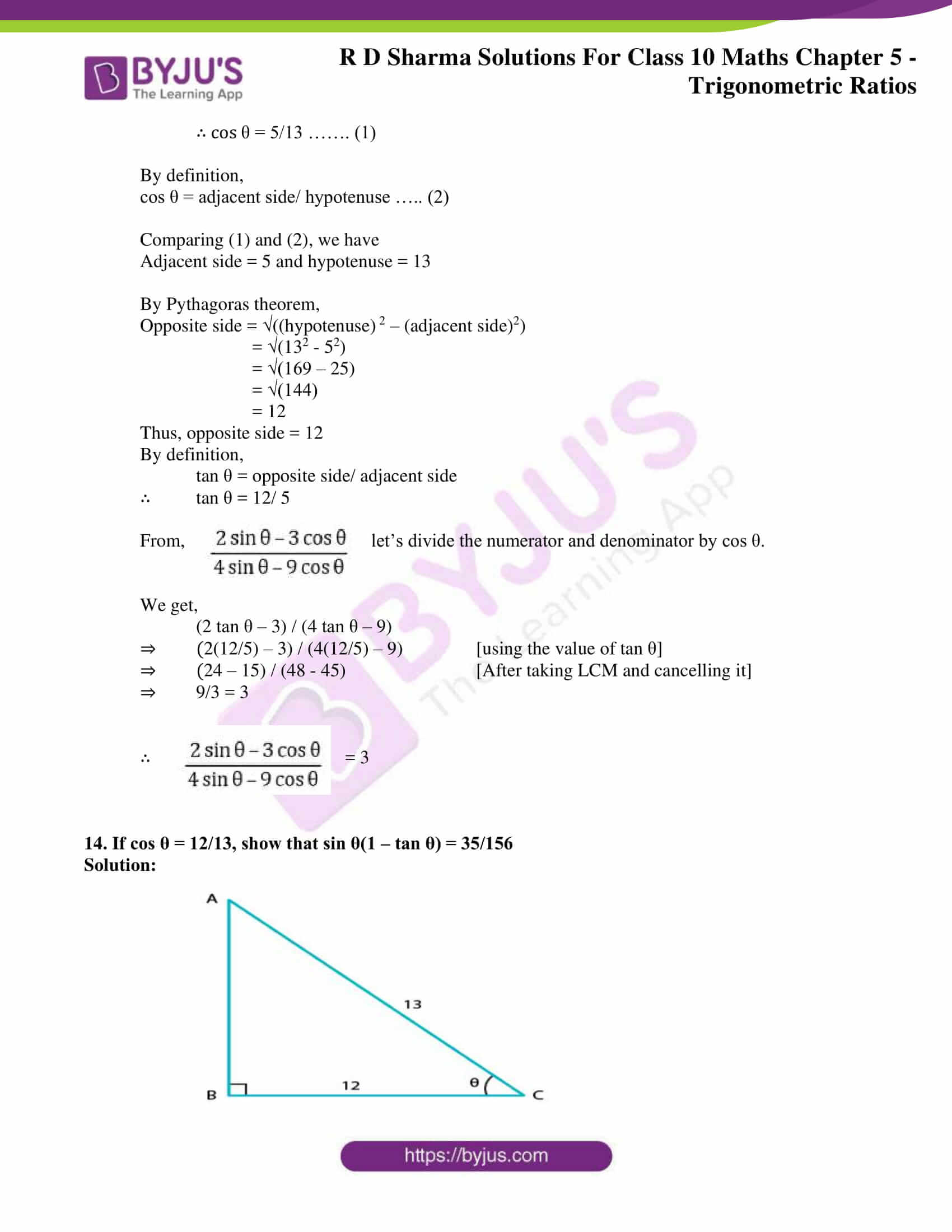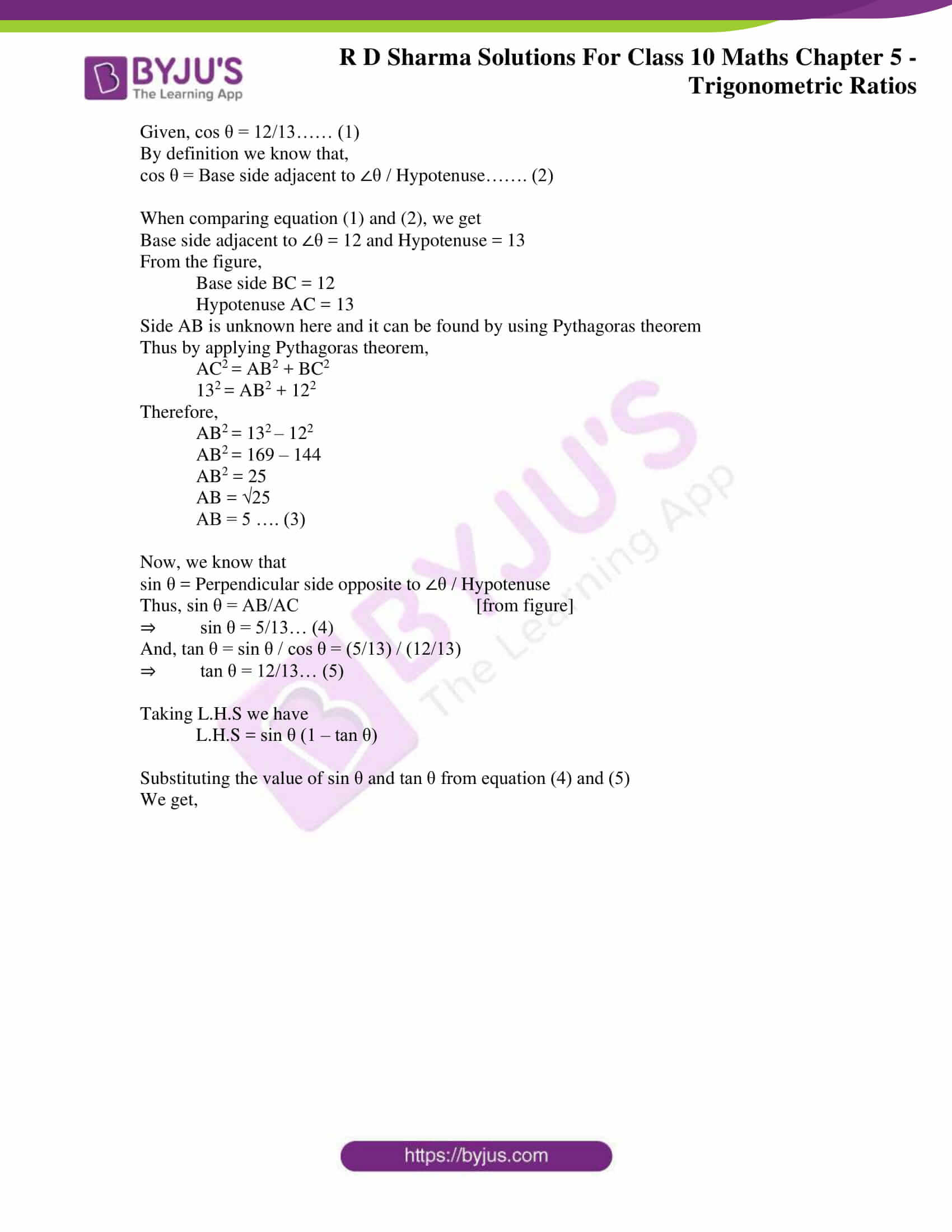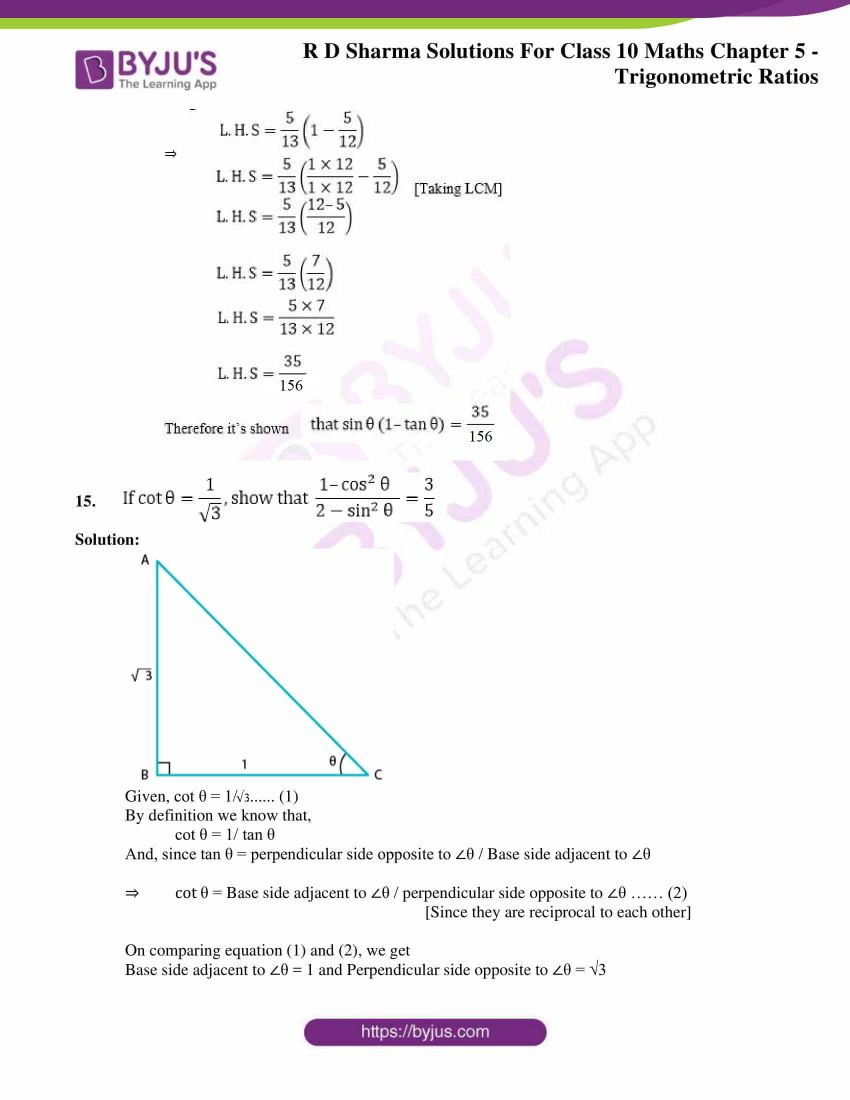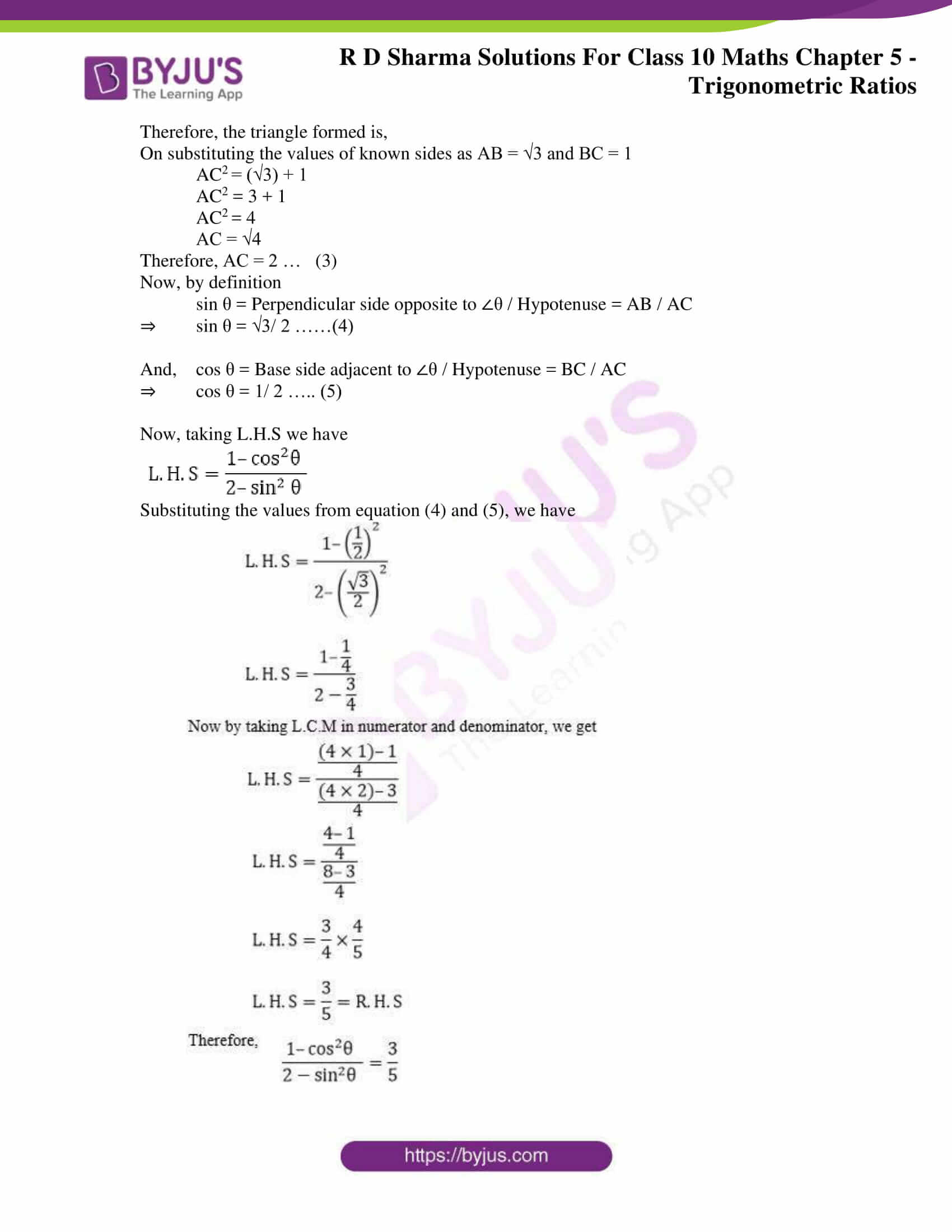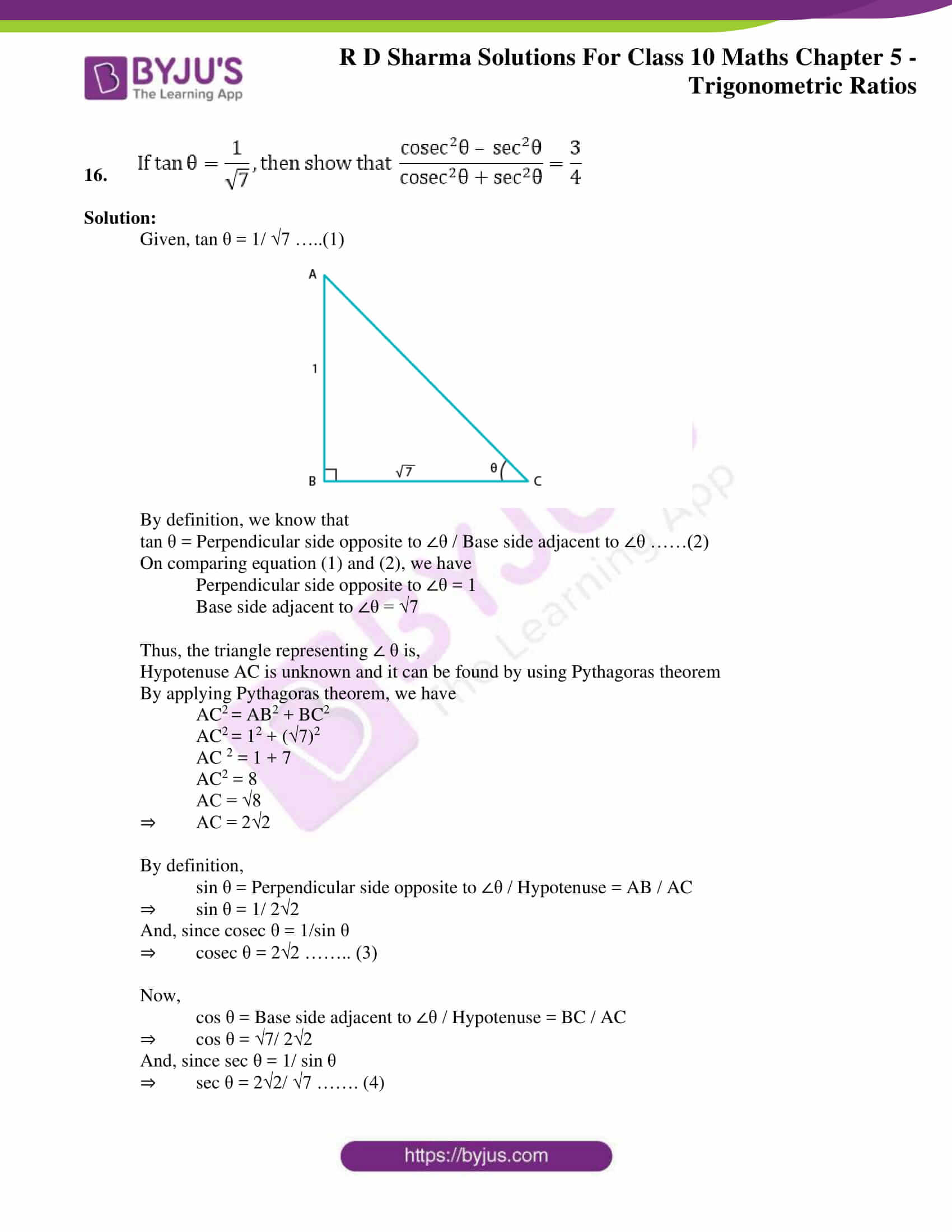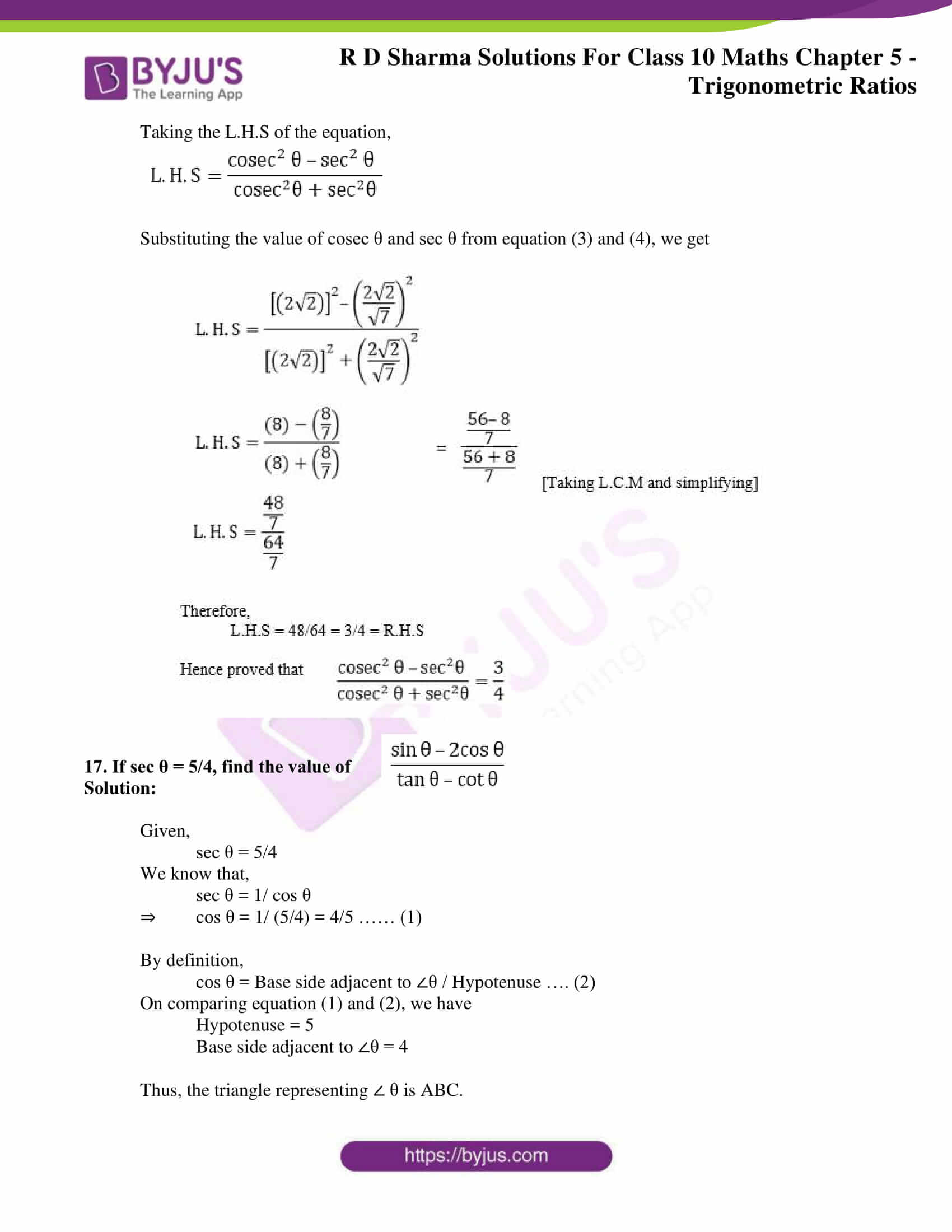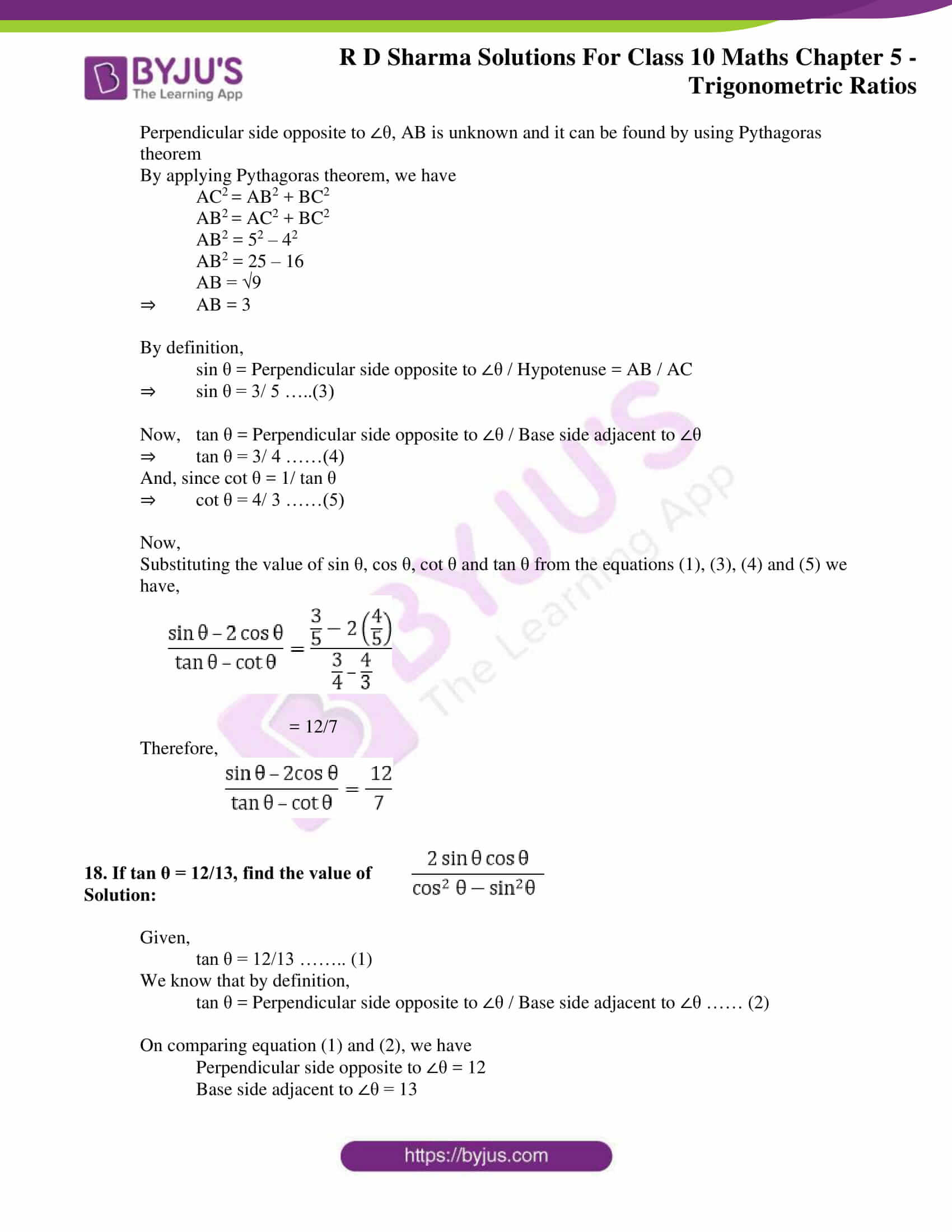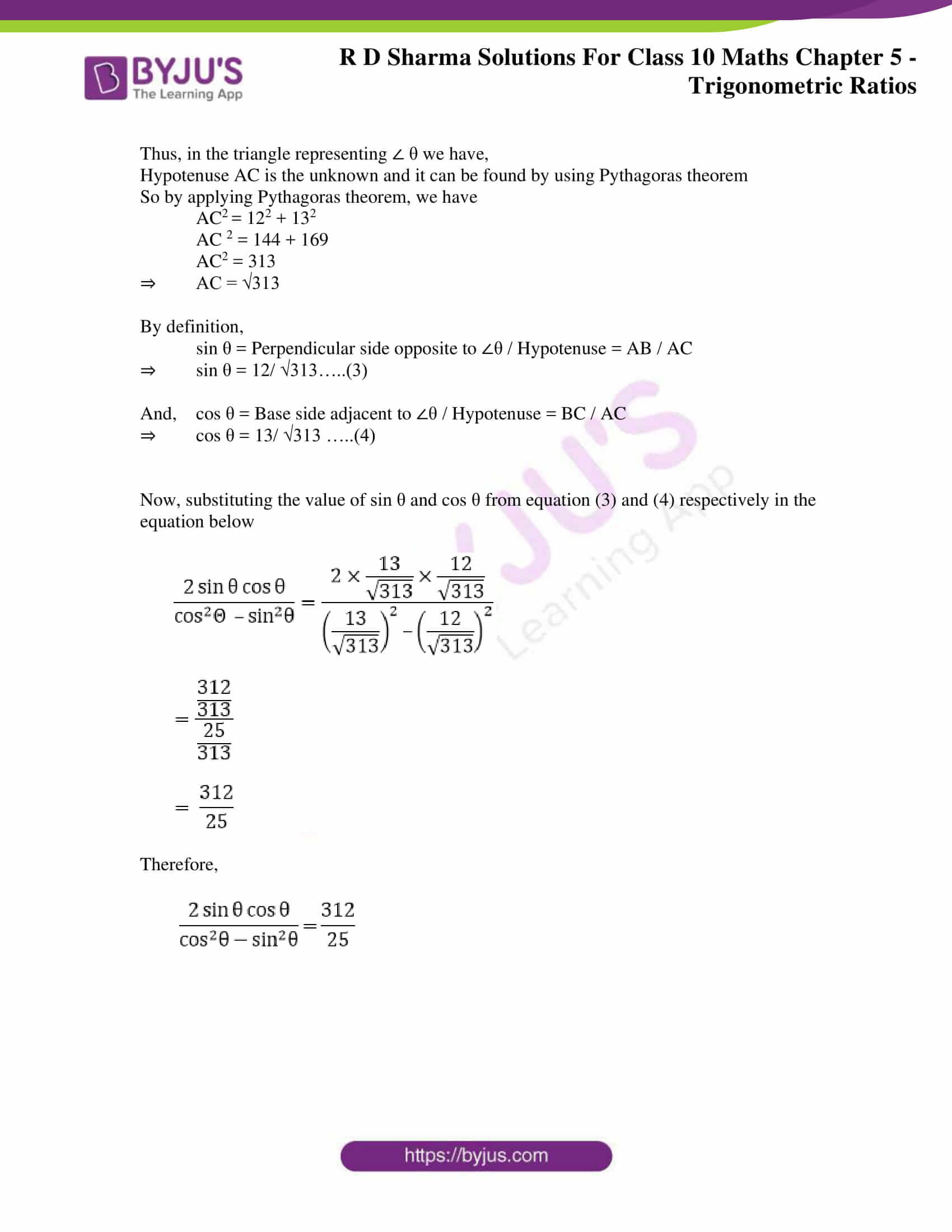### Access RD Sharma Solutions for Class 10 Maths Chapter 5 Trigonometric Ratios Exercise 5.1

1. In each of the following, one of the six trigonometric ratios s given. Find the values of the other trigonometric ratios.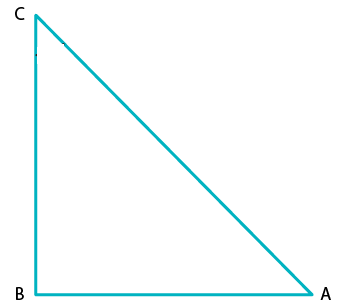(i) sin A = 2/3

Solution:

We have,

sin A = 2/3 ……..….. (1)

As we know, by sin definition;

sin A =  Perpendicular/ Hypotenuse = 2/3 ….(2)

By comparing eq. (1) and (2), we have

Opposite side = 2 and Hypotenuse = 3

Now, on using Pythagoras theorem in Δ ABC

AC= AB2 + BC2

Putting the values of perpendicular side (BC) and hypotenuse (AC) and for the base side as (AB), we get

⇒ 32 = AB2 + 22

AB= 32 – 22

AB2 = 9 – 4

AB2 = 5

AB = √5

Hence, Base = √5

By definition,

cos A = Base/Hypotenuse

⇒ cos A = √5/3

Since, cosec A = 1/sin A = Hypotenuse/Perpendicular

⇒ cosec A = 3/2

And, sec A = Hypotenuse/Base

⇒ sec A = 3/√5

And, tan A = Perpendicular/Base

⇒ tan A =  2/√5

And, cot A = 1/ tan A = Base/Perpendicular

⇒ cot A = √5/2

(ii) cos A = 4/5

Solution:

We have,

cos A = 4/5 …….…. (1)

As we know, by cos defination

cos A = Base/Hypotenuse …. (2)

By comparing eq. (1) and (2), we get

Base = 4 and Hypotenuse = 5

Now, using Pythagoras theorem in Δ ABC

AC= AB+ BC2

Putting the value of base (AB) and hypotenuse (AC) and for the perpendicular (BC), we get

52 = 42 + BC2

BC2 = 52 – 42

BC2 = 25 – 16

BC2 = 9

BC= 3

Hence, Perpendicular = 3

By definition,

sin A = Perpendicular/Hypotenuse

⇒ sin A = 3/5

Then, cosec A = 1/sin A

⇒ cosec A= 1/ (3/5) = 5/3 = Hypotenuse/Perependicular

And, sec A = 1/cos A

⇒ sec A =Hypotenuse/Base

sec A = 5/4

And, tan A = Perpendicular/Base

⇒ tan A = 3/4

Next, cot A = 1/tan A = Base/Perpendicular

∴ cot A = 4/3

(iii) tan θ = 11/1

Solution:

We have, tan θ = 11…..…. (1)

By definition,

tan θ = Perpendicular/ Base…. (2)

On Comparing eq. (1) and (2), we get;

Base = 1 and Perpendicular = 5

Now, using Pythagoras theorem in Δ ABC.

AC2 = AB2 + BC2

Putting the value of base (AB) and perpendicular (BC) to get hypotenuse(AC), we get;

AC2 = 12 + 112

AC2 = 1 + 121

AC2= 122

AC= √122

Hence, hypotenuse = √122

By definition,

sin = Perpendicular/Hypotenuse

⇒ sin θ = 11/√122

And, cosec θ = 1/sin θ

⇒ cosec θ = √122/11

Next, cos θ = Base/ Hypotenuse

⇒ cos θ = 1/√122

And, sec θ = 1/cos θ

⇒ sec θ = √122/1 = √122

And, cot θ  = 1/tan θ

∴ cot θ = 1/11

(iv) sin θ = 11/15

Solution:

We have,  sin θ = 11/15 ………. (1)

By definition,

sin θ = Perpendicular/ Hypotenuse …. (2)

On Comparing eq. (1) and (2), we get;

Perpendicular = 11 and Hypotenuse= 15

Now, using Pythagoras theorem in Δ ABC

AC2 = AB2 + BC2

Putting the value of perpendicular (BC) and hypotenuse (AC) to get the base (AB), we have

152 = AB2 +112

AB2 = 15– 112

AB2 = 225 – 121

AB= 104

AB = √104

AB= √ (2×2×2×13)

AB= 2√(2×13)

AB= 2√26

Hence, Base = 2√26

By definition,

cos θ = Base/Hypotenuse

∴ cosθ = 2√26/ 15

And, cosec θ = 1/sin θ

∴ cosec θ = 15/11

And, secθ = Hypotenuse/Base

∴ secθ =15/ 2√26

And,  tan θ = Perpendicular/Base

∴ tanθ =11/ 2√26

And,  cot θ = Base/Perpendicular

∴ cotθ =2√26/ 11

(v) tan α = 5/12

Solution:

We have,  tan α = 5/12 …. (1)

By definition,

tan α = Perpendicular/Base…. (2)

On Comparing eq. (1) and (2), we get

Base = 12 and Perpendicular side = 5

Now, using Pythagoras theorem in Δ ABC

AC2 = AB2 + BC2

Putting the value of base (AB) and the perpendicular (BC) to get hypotenuse (AC), we have

AC2 = 122 + 52

AC2 = 144 + 25

AC2= 169

AC = 13 [After taking sq root on both sides]

Hence, Hypotenuse = 13

By definition,

sin α  = Perpendicular/Hypotenuse

∴ sin α = 5/13

And, cosec α  = Hypotenuse/Perpendicular

∴ cosec α = 13/5

And,  cos α = Base/Hypotenuse

∴ cos α = 12/13

And,  sec α =1/cos α

∴ sec α = 13/12

And, tan α = sin α/cos α

∴ tan α=5/12

Since, cot α = 1/tan α

∴ cot α =12/5

(vi) sin θ = √3/2

Solution:

We have, sin θ = √3/2 …………. (1)

By definition,

sin θ = Perpendicular/ Hypotenuse….(2)

On Comparing eq. (1) and (2), we get;

Perpendicular = √3 and Hypotenuse = 2

Now, using Pythagoras theorem in Δ ABC

AC2 = AB2 + BC2

Putting the value of perpendicular (BC) and hypotenuse (AC) and get the base (AB), we get;

22 = AB2 + (√3)2

AB2 = 22 – (√3)2

AB2 = 4 – 3

AB2 = 1

AB = 1

Thus, Base = 1

By definition,

cos θ = Base/Hypotenuse

∴ cos θ = 1/2

And, cosec θ = 1/sin θ

Or cosec θ= Hypotenuse/Perpendicualar

∴ cosec θ =2/√3

And,  sec θ = Hypotenuse/Base

∴ sec θ = 2/1

And,  tan θ = Perpendicula/Base

∴ tan θ = √3/1

And,  cot θ = Base/Perpendicular

∴ cot θ = 1/√3

(vii) cos θ = 7/25

Solution:

We have, cos θ = 7/25 ……….. (1)

By definition,

cos θ = Base/Hypotenuse

On Comparing eq. (1) and (2), we get;

Base = 7 and Hypotenuse = 25

Now, using Pythagoras theorem in Δ ABC

AC2= AB2 + BC2

Putting the value of base (AB) and hypotenuse (AC) to get the perpendicular (BC)

252 = 7+BC2

BC2 = 252 – 72

BC2 = 625 – 49

BC2 = 576

BC= √576

BC= 24

Hence, Perpendicular side = 24

By definition,

sin θ = perpendicular/Hypotenuse

∴ sin θ = 24/25

Since, cosec θ = 1/sin θ

Also, cosec θ= Hypotenuse/Perpendicualar

∴ cosec θ = 25/24

Since,  sec θ = 1/cosec θ

Also,  sec θ = Hypotenuse/Base

∴ sec θ = 25/7

Since,  tan θ = Perpendicular/Base

Now,  cot = 1/tan θ

So,  cot θ = Base/Perpendicular

∴ cot θ = 7/24

(viii) tan θ = 8/15

Solution:

We have, tan θ = 8/15 …………. (1)

By definition,

tan θ = Perpendicular/Base …. (2)

On Comparing eq. (1) and (2), we get;

Base = 15 and Perpendicular = 8

Now, using Pythagoras theorem in Δ ABC

AC2 = 152 + 82

AC2 = 225 + 64

AC2 = 289

AC = √289

AC = 17

Hence, Hypotenuse = 17

By definition,

Since,  sin θ = perpendicular/Hypotenuse

∴ sin θ = 8/17

Since, cosec θ = 1/sin θ

Also, cosec θ = Hypotenuse/Perpendicualar

∴ cosec θ = 17/8

Since,  cos θ = Base/Hypotenuse

∴ cos θ = 15/17

Since,  sec θ = 1/cos θ

Also,  sec θ = Hypotenuse/Base

∴ sec θ = 17/15

Since, cot θ = 1/tan θ

Also,  cot θ = Base/Perpendicular

∴ cot θ = 15/8

(ix) cot θ = 12/5

Solution:

We have, cot θ = 12/5 …………. (1)

By definition,

cot θ = 1/tan θ

cot θ = Base/Perpendicular ……. (2)

On Comparing eq. (1) and (2), we have

Base = 12 and Perpendicular side = 5

Now, using Pythagoras theorem in Δ ABC

AC2= AB2 + BC2

Putting the value of base (AB) and perpendicular (BC) to get the hypotenuse (AC);

AC2 = 122 + 52

AC2= 144 + 25

AC2 = 169

AC = √169

AC = 13

Hence, Hypotenuse = 13

By definition,

Since,  sin θ = perpendicular/Hypotenuse

∴ sin θ= 5/13

Since, cosec θ = 1/sin θ

Also, cosec θ= Hypotenuse/Perpendicualar

∴ cosec θ = 13/5

Since,  cos θ = Base/Hypotenuse

∴ cos θ = 12/13

Since,  sec θ = 1/cosθ

Also,  sec θ = Hypotenuse/Base

∴ sec θ = 13/12

Since,  tanθ = 1/cot θ

Also,  tan θ = Perpendicular/Base

∴ tan θ = 5/12

(x)  sec θ = 13/5

Solution:

We have, sec θ = 13/5…….… (1)

By definition,

sec θ = Hypotenuse/Base…………. (2)

On Comparing eq. (1) and (2), we get

Base = 5 and Hypotenuse = 13

Now, using Pythagoras theorem in Δ ABC

AC= AB2 + BC2

And. putting the value of base side (AB) and hypotenuse (AC) to get the perpendicular side (BC)

132 = 52 + BC2

BC2 = 13– 52

BC2=169 – 25

BC2= 144

BC= √144

BC = 12

Hence, Perpendicular = 12

By definition,

Since,  sin θ = perpendicular/Hypotenuse

∴ sin θ= 12/13

Since, cosec θ= 1/ sin θ

Also, cosec θ= Hypotenuse/Perpendicualar

∴ cosec θ = 13/12

Since,  cos θ= 1/sec θ

Also,  cos θ = Base/Hypotenuse

∴ cos θ = 5/13

Since,  tan θ = Perpendicular/Base

∴ tan θ = 12/5

Since,  cot θ = 1/tan θ

Also,  cot θ = Base/Perpendicular

∴ cot θ = 5/12

(xi)  cosec θ = √10

Solution:

We have, cosec θ = √10/1   ……..… (1)

By definition,

cosec θ = Hypotenuse/ Perpendicualar …….….(2)

And, cosecθ = 1/sin θ

On comparing eq.(1) and(2), we get

Perpendicular side = 1 and Hypotenuse = √10

Now, using Pythagoras theorem in Δ ABC

AC= AB2 + BC2

Putting the value of perpendicular (BC) and hypotenuse (AC) to get the base side (AB)

(√10)2 = AB2 + 12

AB2= (√10)2 – 12

AB2= 10 – 1

AB = √9

AB = 3

So, Base side = 3

By definition,

Since,  sin θ = Perpendicular/Hypotenuse

∴ sin θ = 1/√10

Since,  cos θ = Base/Hypotenuse

∴ cos θ = 3/√10

Since,  sec θ = 1/cos θ

Also, sec θ = Hypotenuse/Base

∴ sec θ = √10/3

Since,  tan θ = Perpendicular/Base

∴ tan θ = 1/3

Since,  cot θ = 1/tan θ

∴ cot θ = 3/1

(xii)  cos θ =12/15

Solution:

We have; cos θ = 12/15 ………. (1)

By definition,

cos θ = Base/Hypotenuse……… (2)

By comparing eq. (1) and (2), we get;

Base =12 and Hypotenuse = 15

Now, using Pythagoras theorem in Δ ABC, we get

AC2 = AB2+ BC2

Putting the value of base (AB) and hypotenuse (AC) to get the perpendicular (BC);

152 = 122 + BC2

BC2 = 152 – 122

BC2 = 225 – 144

BC 2= 81

BC = √81

BC = 9

So, Perpendicular = 9

By definition,

Since,  sin θ = perpendicular/Hypotenuse

∴ sin θ = 9/15 = 3/5

Since, cosec θ = 1/sin θ

Also, cosec θ = Hypotenuse/Perpendicualar

∴ cosec θ= 15/9 = 5/3

Since,  sec θ = 1/cos θ

Also,  sec θ = Hypotenuse/Base

∴ sec θ = 15/12 = 5/4

Since,  tan θ = Perpendicular/Base

∴ tan θ = 9/12 = 3/4

Since,  cot θ = 1/tan θ

Also,  cot θ = Base/Perpendicular

∴ cot θ = 12/9 = 4/3

2. In a △ ABC, right angled at B, AB = 24 cm , BC = 7 cm. Determine

(i) sin A , cos A (ii) sin C, cos C

Solution: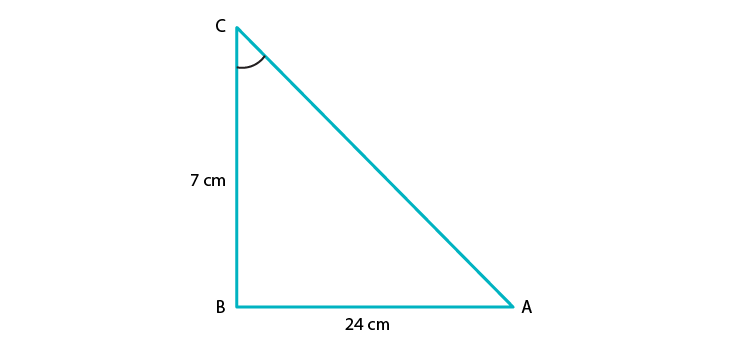(i) Given: In △ABC, AB = 24 cm, BC = 7cm and ∠ABC = 90o

To find: sin A, cos A

By using Pythagoras theorem in △ABC we have

AC2 = AB2 + BC2

AC2 = 242 + 72

AC2 = 576 + 49

AC2= 625

AC = √625

AC= 25

Hence, Hypotenuse = 25

By definition,

sin A = Perpendicular side opposite to angle A/ Hypotenuse

sin A = BC/ AC

sin A = 7/ 25

And,

cos A = Base side adjacent to angle A/Hypotenuse

cos A = AB/ AC

cos A = 24/ 25

(ii) Given: In △ABC , AB = 24 cm and BC = 7cm and ∠ABC = 90o

To find: sin C, cos C

By using Pythagoras theorem in △ABC we have

AC2 = AB2 + BC2

AC2 = 242 + 72

AC2 = 576 + 49

AC2= 625

AC = √625

AC= 25

Hence, Hypotenuse = 25

By definition,

sin C = Perpendicular side opposite to angle C/Hypotenuse

sin C = AB/ AC

sin C = 24/ 25

And,

cos C = Base side adjacent to angle C/Hypotenuse

cos A = BC/AC

cos A = 7/25

3. In fig. 5.37, find tan P and cot R. Is tan P = cot R?

Solution: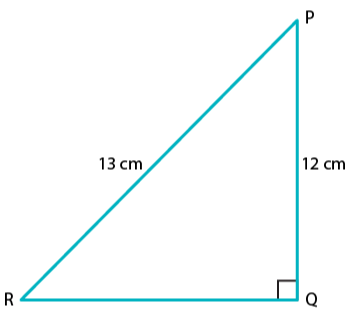By using Pythagoras theorem in △PQR, we have

PR2 = PQ2 + QR2

Putting the length of given side PR and PQ in the above equation

132 = 122 + QR2

QR2 = 132 – 122

QR2 = 169 – 144

QR2 = 25

QR = √25 = 5

By definition,

tan P = Perpendicular side opposite to P/ Base side adjacent to angle P

tan P = QR/PQ

tan P = 5/12 ………. (1)

And,

cot R= Base/Perpendicular

cot R= QR/PQ

cot R= 5/12 …. (2)

When comparing equation (1) and (2), we can see that R.H.S of both the equation is equal.

Therefore, L.H.S of both equations should also be equal.

∴ tan P = cot R

Yes, tan P = cot R = 5/12

4. If sin A = 9/41, compute cos A and tan A.

Solution: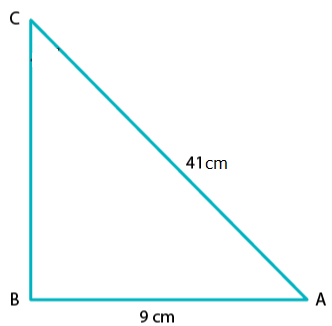Given that, sin A = 9/41 …………. (1)

Required to find: cos A, tan A

By definition, we know that

sin A = Perpendicular/ Hypotenuse……………(2)

On Comparing eq. (1) and (2), we get;

Perpendicular side = 9 and Hypotenuse = 41

Let’s construct △ABC as shown below,

And, here the length of base AB is unknown.

Thus, by using Pythagoras theorem in △ABC, we get;

AC2 = AB2 + BC2

412 = AB2 + 92

AB2 = 412 – 92

AB2 = 168 – 81

AB= 1600

AB = √1600

AB = 40

⇒ Base of triangle ABC, AB = 40

We know that,

cos A = Base/ Hypotenuse

cos A =AB/AC

cos A =40/41

And,

tan A = Perpendicular/ Base

tan A = BC/AB

tan A = 9/40

5.  Given 15cot A= 8, find sin A and sec A.

Solution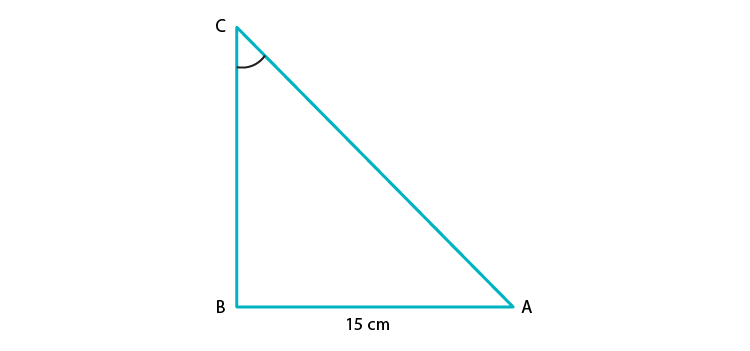We have, 15cot A = 8

Required to find: sin A and sec A

As, 15 cot A = 8

⇒ cot A = 8/15 …….(1)

And we know,

cot A = 1/tan A

Also by definition,

Cot A = Base side adjacent to ∠A/ Perpendicular side opposite to ∠A …. (2)

On comparing equation (1) and (2), we get;

Base side adjacent to ∠A = 8

Perpendicular side opposite to ∠A = 15

So, by using Pythagoras theorem to △ABC, we have

AC2 = AB2 +BC2

Substituting values for sides from the figure

AC2 = 82 + 152

AC2 = 64 + 225

AC2 = 289

AC = √289

AC = 17

Therefore, hypotenuse =17

By definition,

sin A = Perpendicular/Hypotenuse

⇒ sin A= BC/AC

sin A= 15/17 (using values from the above)

Also,

sec A= 1/ cos A

⇒ secA = Hypotenuse/ Base side adjacent to ∠A

∴ sec A= 17/8

6. In △PQR, right-angled at Q, PQ = 4cm and RQ = 3 cm. Find the value of sin P, sin R, sec P and sec R.

Solution: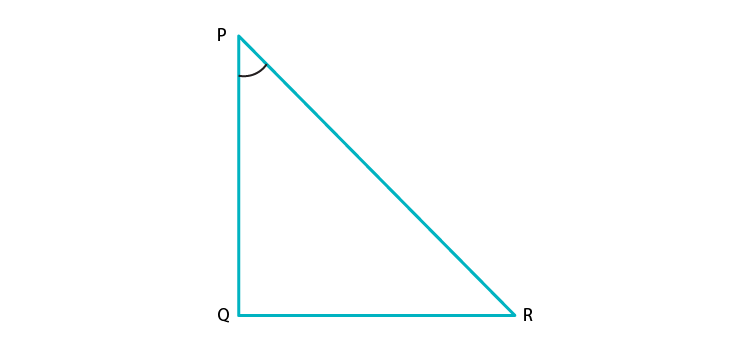Given:

△PQR is right-angled at Q.

PQ = 4cm

RQ = 3cm

Required to find: sin P, sin R, sec P, sec R

Given △PQR,

By using Pythagoras theorem to △PQR, we get

PR2 = PQ2 +RQ2

Substituting the respective values,

PR2 = 42 +32

PR2 = 16 + 9

PR2 = 25

PR = √25

PR = 5

⇒ Hypotenuse =5

By definition,

sin P = Perpendicular side opposite to angle P/ Hypotenuse

sin P = RQ/ PR

⇒ sin P = 3/5

And,

sin R = Perpendicular side opposite to angle R/ Hypotenuse

sin R = PQ/ PR

⇒ sin R = 4/5

And,

sec P=1/cos P

secP = Hypotenuse/ Base side adjacent to ∠P

sec P = PR/ PQ

⇒ sec P = 5/4

Now,

sec R = 1/cos R

secR = Hypotenuse/ Base side adjacent to ∠R

sec R = PR/ RQ

⇒ sec R = 5/3

7. If cot θ = 7/8, evaluate

(i)   (1+sin θ)(1–sin θ)/ (1+cos θ)(1–cos θ)

(ii)  cot2 θ

Solution:

(i) Required to evaluate: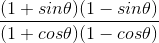, given = cot θ = 7/8

Taking the numerator, we have

(1+sin θ)(1–sin θ) = 1 – sin2 θ [Since, (a+b)(a-b) = a2 – b2]

Similarly,

(1+cos θ)(1–cos θ) = 1 – cos2 θ

We know that,

sin2 θ + cos2 θ = 1

⇒ 1 – cos2 θ = sin2 θ

And,

1 – sin2 θ = cos2 θ

Thus,

(1+sin θ)(1 –sin θ) = 1 – sin2 θ = cos2 θ

(1+cos θ)(1–cos θ) = 1 – cos2 θ = sin2 θ= cos2 θ/ sin2 θ

= (cos θ/sin θ)2

And, we know that (cos θ/sin θ) = cot θ= (cot θ)2

= (7/8)2

= 49/ 64

(ii) Given,

cot θ = 7/8

So, by squaring on both sides we get

(cot θ)2 = (7/8)2

∴ cot θ2 = 49/64

8. If 3cot A = 4, check whether (1–tan2A)/(1+tan2A) = (cos2A – sin2A) or not.

Solution: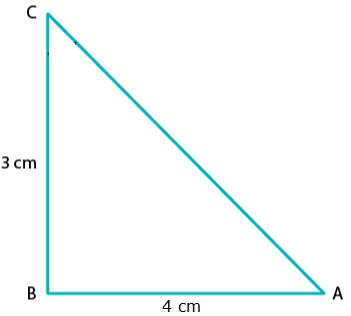Given,

3cot A = 4

⇒ cot A = 4/3

By definition,

tan A = 1/ Cot A = 1/ (4/3)

⇒ tan A = 3/4

Thus,

Base side adjacent to ∠A = 4

Perpendicular side opposite to ∠A = 3

In ΔABC, Hypotenuse is unknown

Thus, by applying Pythagoras theorem in ΔABC

We get

AC= AB2 + BC2

AC2 = 42 + 32

AC2 = 16 + 9

AC2 = 25

AC = √25

AC = 5

Hence, hypotenuse = 5

Now, we can find that

sin A = opposite side to ∠A/ Hypotenuse = 3/5

And,

cos A = adjacent side to ∠A/ Hypotenuse = 4/5

Taking the LHS,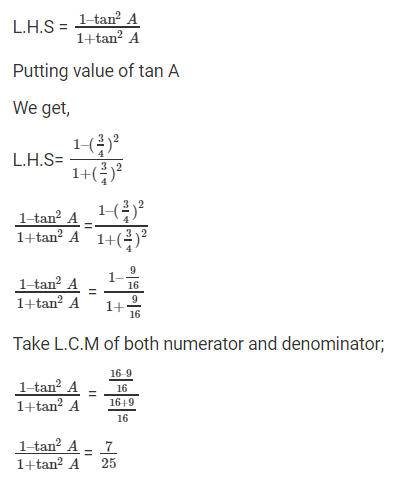Thus, LHS = 7/25

Now, taking RHS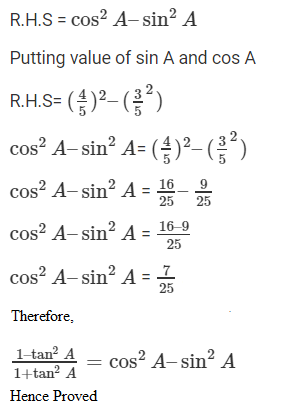9. If tan θ = a/b, find the value of (cos θ + sin θ)/ (cos θ – sin θ)

Solution:

Given,

tan θ = a/b

And, we know by definition that

tan θ = opposite side/ adjacent side

Thus, by comparison

Opposite side = a and adjacent side = b

To find the hypotenuse, we know that by Pythagoras theorem that

Hypotenuse2 = opposite side2 + adjacent side2

⇒ Hypotenuse = √(a2 + b2)

So, by definition

sin θ = opposite side/ Hypotenuse

sin θ = a/ √(a2 + b2)

And,

cos θ = adjacent side/ Hypotenuse

cos θ = b/ √(a2 + b2)

Now,

After substituting for cos θ and sin θ, we have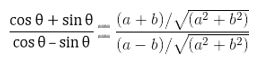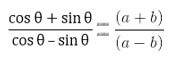Hence Proved.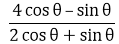10. If 3 tan θ = 4, find the value of

Solution:

Given, 3 tan θ = 4

⇒ tan θ = 4/3From, let’s divide the numerator and denominator by cos θ.

We get,

(4 – tan θ) / (2 + tan θ)

⇒ (4 – (4/3)) / (2 + (4/3)) [using the value of tan θ]

⇒ (12 – 4) / (6 + 4) [After taking LCM and cancelling it]

⇒ 8/10 = 4/5∴ = 4/5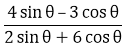11. If 3 cot θ = 2, find the value of

Solution:

Given, 3 cot θ = 2

⇒ cot θ = 2/3From, let’s divide the numerator and denominator by sin θ.

We get,

(4 –3 cot θ) / (2 + 6 cot θ)

⇒ (4 – 3(2/3)) / (2 + 6(2/3)) [using the value of tan θ]

⇒ (4 – 2) / (2 + 4) [After taking LCM and simplifying it]

⇒ 2/6 = 1/3∴ = 1/3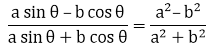12. If tan θ = a/b, prove that

Solution:

Given, tan θ = a/b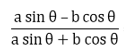From LHS, let’s divide the numerator and denominator by cos θ.

And we get,

(a tan θ – b) / (a tan θ + b)

⇒ (a(a/b) – b) / (a(a/b) + b) [using the value of tan θ]

⇒ (a2 – b2)/b2 / (a2 + b2)/b2 [After taking LCM and simplifying it]

⇒ (a2 – b2)/ (a2 + b2)

= RHS

– Hence Proved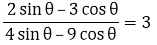13. If sec θ = 13/5, show that

Solution: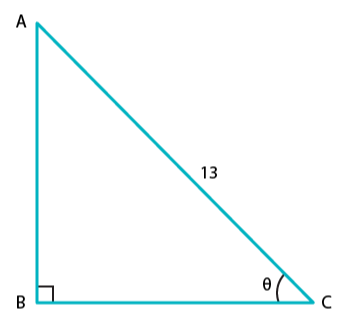Given,

sec θ = 13/5

We know that,

sec θ = 1/ cos θ

⇒ cos θ = 1/ sec θ = 1/ (13/5)

∴ cos θ = 5/13 ……. (1)

By definition,

cos θ = adjacent side/ hypotenuse ….. (2)

Comparing (1) and (2), we have

Adjacent side = 5 and hypotenuse = 13

By Pythagoras theorem,

Opposite side = √((hypotenuse) 2 – (adjacent side)2)

= √(132 – 52)

= √(169 – 25)

= √(144)

= 12

Thus, opposite side = 12

By definition,

tan θ = opposite side/ adjacent side

∴ tan θ = 12/ 5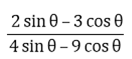From, let’s divide the numerator and denominator by cos θ.

We get,

(2 tan θ – 3) / (4 tan θ – 9)

⇒ (2(12/5) – 3) / (4(12/5) – 9) [using the value of tan θ]

⇒ (24 – 15) / (48 – 45) [After taking LCM and cancelling it]

⇒ 9/3 = 3∴ = 3

14. If cos θ = 12/13, show that sin θ(1 – tan θ) = 35/156

Solution: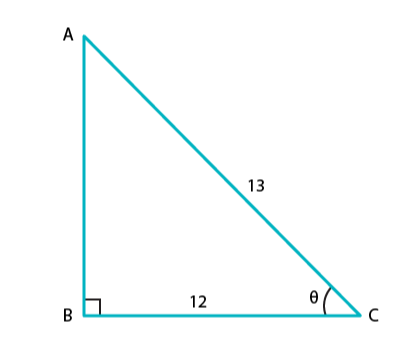Given, cos θ = 12/13…… (1)

By definition we know that,

cos θ = Base side adjacent to ∠θ / Hypotenuse……. (2)

When comparing equation (1) and (2), we get

Base side adjacent to ∠θ = 12 and Hypotenuse = 13

From the figure,

Base side BC = 12

Hypotenuse AC = 13

Side AB is unknown here and it can be found by using Pythagoras theorem

Thus by applying Pythagoras theorem,

AC2 = AB2 + BC2

132 = AB2 + 122

Therefore,

AB2 = 13– 122

AB2 = 169 – 144

AB2 = 25

AB = √25

AB = 5 …. (3)

Now, we know that

sin θ = Perpendicular side opposite to ∠θ / Hypotenuse

Thus, sin θ = AB/AC [from figure]

⇒ sin θ = 5/13… (4)

And, tan θ = sin θ / cos θ = (5/13) / (12/13)

⇒ tan θ = 12/13… (5)

Taking L.H.S we have

L.H.S = sin θ (1 – tan θ)

Substituting the value of sin θ and tan θ from equation (4) and (5)

We get,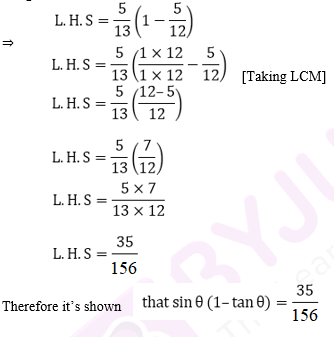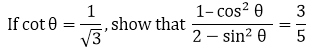15.

Solution: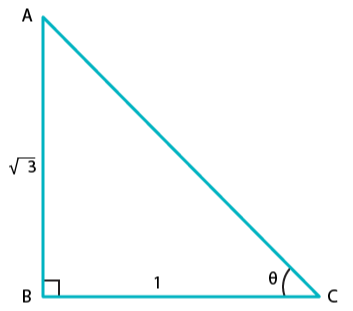Given, cot θ = 1/3……. (1)

By definition we know that,

cot θ = 1/ tan θ

And, since tan θ = perpendicular side opposite to ∠θ / Base side adjacent to ∠θ

⇒ cot θ = Base side adjacent to ∠θ / perpendicular side opposite to ∠θ …… (2)

[Since they are reciprocal to each other]

On comparing equation (1) and (2), we get

Base side adjacent to ∠θ = 1 and Perpendicular side opposite to ∠θ = √3

Therefore, the triangle formed is,

On substituting the values of known sides as AB = √3 and BC = 1

AC2 = (√3) + 1

AC2 = 3 + 1

AC= 4

AC = √4

Therefore, AC = 2 …   (3)

Now, by definition

sin θ = Perpendicular side opposite to ∠θ / Hypotenuse = AB / AC

⇒ sin θ = √3/ 2 ……(4)

And, cos θ = Base side adjacent to ∠θ / Hypotenuse = BC / AC

⇒ cos θ = 1/ 2 ….. (5)

Now, taking L.H.S we have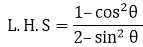Substituting the values from equation (4) and (5), we have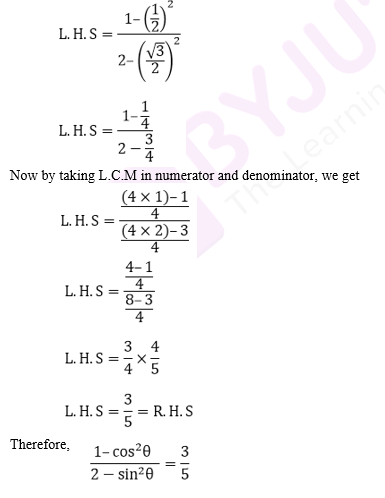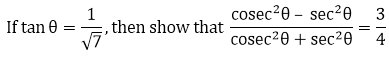16.

Solution:

Given, tan θ = 1/ √7 …..(1)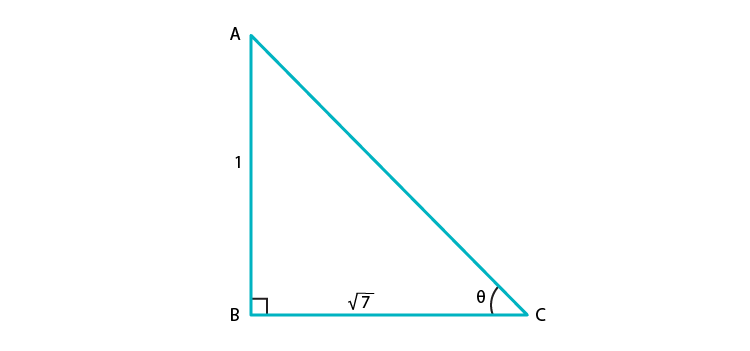By definition, we know that

tan θ = Perpendicular side opposite to ∠θ / Base side adjacent to ∠θ ……(2)

On comparing equation (1) and (2), we have

Perpendicular side opposite to ∠θ = 1

Base side adjacent to ∠θ = √7

Thus, the triangle representing ∠ θ is,

Hypotenuse AC is unknown and it can be found by using Pythagoras theorem

By applying Pythagoras theorem, we have

AC= AB2 + BC2

AC= 12 + (√7)2

AC 2 = 1 + 7

AC2 = 8

AC = √8

⇒ AC = 2√2

By definition,

sin θ = Perpendicular side opposite to ∠θ / Hypotenuse = AB / AC

⇒ sin θ = 1/ 2√2

And, since cosec θ = 1/sin θ

⇒ cosec θ = 2√2 …….. (3)

Now,

cos θ = Base side adjacent to ∠θ / Hypotenuse = BC / AC

⇒ cos θ = √7/ 2√2

And, since sec θ = 1/ sin θ

⇒ sec θ = 2√2/ √7 ……. (4)

Taking the L.H.S of the equation,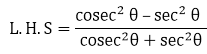Substituting the value of cosec θ and sec θ from equation (3) and (4), we get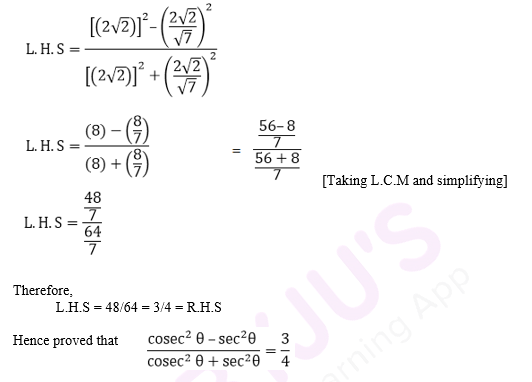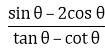17. If sec θ = 5/4, find the value of

Solution:

Given,

sec θ = 5/4

We know that,

sec θ = 1/ cos θ

⇒ cos θ = 1/ (5/4) = 4/5 …… (1)

By definition,

cos θ = Base side adjacent to ∠θ / Hypotenuse …. (2)

On comparing equation (1) and (2), we have

Hypotenuse = 5

Base side adjacent to ∠θ = 4

Thus, the triangle representing ∠ θ is ABC.

Perpendicular side opposite to ∠θ, AB is unknown and it can be found by using Pythagoras theorem

By applying Pythagoras theorem, we have

AC= AB2 + BC2

AB= AC2 + BC2

AB2 = 52 – 42

AB2 = 25 – 16

AB = √9

⇒ AB = 3

By definition,

sin θ = Perpendicular side opposite to ∠θ / Hypotenuse = AB / AC

⇒ sin θ = 3/ 5 …..(3)

Now, tan θ = Perpendicular side opposite to ∠θ / Base side adjacent to ∠θ

⇒ tan θ = 3/ 4 ……(4)

And, since cot θ = 1/ tan θ

⇒ cot θ = 4/ 3 ……(5)

Now,

Substituting the value of sin θ, cos θ, cot θ and tan θ from the equations (1), (3), (4) and (5) we have,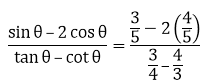= 12/7

Therefore,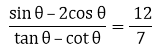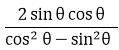18. If tan θ = 12/13, find the value of

Solution:

Given,

tan θ = 12/13 …….. (1)

We know that by definition,

tan θ = Perpendicular side opposite to ∠θ / Base side adjacent to ∠θ …… (2)

On comparing equation (1) and (2), we have

Perpendicular side opposite to ∠θ = 12

Base side adjacent to ∠θ = 13

Thus, in the triangle representing ∠ θ we have,

Hypotenuse AC is the unknown and it can be found by using Pythagoras theorem

So by applying Pythagoras theorem, we have

AC= 122 + 132

AC 2 = 144 + 169

AC2 = 313

⇒ AC = √313

By definition,

sin θ = Perpendicular side opposite to ∠θ / Hypotenuse = AB / AC

⇒ sin θ = 12/ √313…..(3)

And, cos θ = Base side adjacent to ∠θ / Hypotenuse = BC / AC

⇒ cos θ = 13/ √313 …..(4)

Now, substituting the value of sin θ and cos θ from equation (3) and (4) respectively in the equation below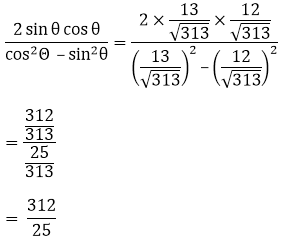Therefore,